• ## Numpy 数据精度

千次阅读 2017-07-06 10:58:04
...双精度11位阶码，1位符号，剩下52位尾数，算出2的负52次方，得到0.00000000000000022204460492503130808472633361816 前面0有多少个，就表示能精确到那一位 参考文献 ...
https://docs.scipy.org/doc/numpy-dev/user/basics.types.html
数据类型描述bool_Boolean (True or False) stored as a byteint_Default integer type (same as C long normally either int64 or int32)intcIdentical to C int (normally int32 or int64)intpInteger used for indexing (same as C ssize_tnormally either int32 or int64)int8Byte (-128 to 127)int16Integer (-32768 to 32767)int32Integer (-2147483648 to 2147483647)int64Integer (-9223372036854775808 to 9223372036854775807)uint8Unsigned integer (0 to 255)uint16Unsigned integer (0 to 65535)uint32Unsigned integer (0 to 4294967295)uint64Unsigned integer (0 to 18446744073709551615)float_Shorthand for float64.float16Half precision float: sign bit, 5 bits exponent, 10 bits mantissafloat32Single precision float: sign bit, 8 bits exponent, 23 bits mantissafloat64Double precision float: sign bit, 11 bits exponent, 52 bits mantissacomplex_Shorthand for complex128.complex64Complex number, represented by two 32-bit floats (real and imaginary components)complex128Complex number, represented by two 64-bit floats (real and imaginary components)
单精度8位阶码，1位符号，剩下23位尾数，算出2的负23次方，得到0.00000011920928955078125  前面0有多少个，就表示能精确到那一位  双精度11位阶码，1位符号，剩下52位尾数，算出2的负52次方，得到0.00000000000000022204460492503130808472633361816  前面0有多少个，就表示能精确到那一位
参考文献  http://bbs.bccn.net/thread-316069-1-1.html  http://blog.csdn.net/qq_34312386/article/details/52454701  http://blog.sina.com.cn/s/blog_814e83d80101bgcf.html  https://zhidao.baidu.com/question/181927800.html
展开全文c语言 数据 boolean class
• import numpy as np &gt;&gt;&gt; t1 = np.arange(0, 0.01*57, 0.01) &gt;&gt;&gt; t1.shape (58L,) 但是如果用同样的方法，可以得到正确的长度为58的、从0开始的、...
1.发现问题
想得到一个长度为57的、从0开始的、间隔为0.01的数组，想当然地如下coding，结果意料之外：
>>> import numpy as np
>>> t1 = np.arange(0, 0.01*57, 0.01)
>>> t1.shape
(58L,)
但是如果用同样的方法，可以得到正确的长度为58的、从0开始的、间隔为0.01的数组，结果如下：
>>> t2 = np.arange(0, 0.01*58, 0.01)
>>> t2.shape
(58L,)
到底是什么出了问题？
2.浮点数的精度问题
查找了一些资料，比较乱，通过总结和整理，列出一些关键内容，以便理解为什么会出现上述问题。
（1）在计算机中用二进制表示小数
python中的浮点型（float）类似于C语言中的double类型（我觉得其实就是一样的，毕竟标准的python是用C写的），是双精度浮点型，可以用直接的十进制或科学计数法表示。每个双精度浮点数占8个字节（64位），完全遵守IEEE754号规范（52M/11E/1S），即其中的52位用于表示底数，11位用于表示指数，剩下一个位表示符号。看上去相当完美，但是实际精度依赖于机器架构和创建python解释器的编译器。我们将0.1表示为二进制的形式，如下计算，可以看到0.1在十进制下明明是一个有限小数，在二进制下表示却是无限循环的（实际上0.0~0.9这十个小数中只有0.0和0.5在二进制形式表示下是有限的）：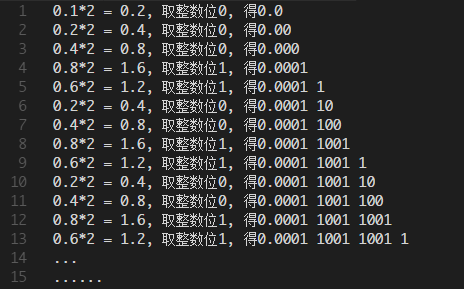根据IEEE754标准，有效位数只能表示52位，在python下，0.1其实是这样表示的（python没有提供.bin函数，只有.hex函数）： >>> (0.1).hex()
'0x1.999999999999ap-4'0x表示十六进制，p-4表示小数点要向左移动4位；IEEE754标准中的52位在这里是不包括小数点前面的那个1的，所以将'0x1.999999999999ap-4'展开，结果如下： 
# 原表示：

0.0001 1001 1001 1001 1001 1001 1001 1001 1001 1001 1001 1001 1001 1001 1001 ···

# IEEE754标准下的表示：

0.0001 1001 1001 1001 1001 1001 1001 1001 1001 1001 1001 1001 1001 1010可以看到，由于计算机存储的结构限制，在IEEE754标准下，本该无限循环的0.1被“1进”了（“0舍1进”，类似于十进制中的“四舍五入”）
（2）IEEE754标准下浮点数精度带来的问题
在涉及精度计算的过程中产生问题
python >>> print '%.20f'%(0.1)
0.10000000000000000555
>>> print '%.20f'%(0.1+0.1)
0.20000000000000001110
>>> print '%.20f'%(0.2)
0.20000000000000001110
>>> print '%.20f'%(0.1+0.2)
0.30000000000000004441
>>> print '%.20f'%(0.1*3)
0.30000000000000004441
>>> print '%.20f'%(0.3)
0.29999999999999998890C++ D:\develop
λ vi test.cpp

#include<iostream>

#include<iomanip>

using namespace std;
int main()
{
double a=0.1,b=0.2,c=0.3;
int d=3;
cout<<"0.1 =     "<<setprecision(20)<<a<<endl;
cout<<"0.1+0.1 = "<<setprecision(20)<<a+a<<endl;
cout<<"0.2 =     "<<setprecision(20)<<b<<endl;
cout<<"0.1+0.2 = "<<setprecision(20)<<a+b<<endl;
cout<<"0.1*3 =   "<<setprecision(20)<<a*d<<endl;
cout<<"0.3 =     "<<setprecision(20)<<c<<endl;
}

:wq

D:\develop
λ g++ test.cpp -o test.exe && .\test.exe
0.1 =     0.10000000000000000555
0.1+0.1 = 0.2000000000000000111
0.2 =     0.2000000000000000111
0.1+0.2 = 0.30000000000000004441
0.1*3 =   0.30000000000000004441
0.3 =     0.2999999999999999889 可以看到，C++和python的情况是一致的。  在编程语言上的0.3实际是比数学上的0.3要小一点点的；而0.1+0.2或0.1*3运算，在编程语言上的误差会跟着进行运算，运算结果会积累误差造成其要比数学上的0.3要大一点点。 解析numpy.arange使用浮点数的问题  根据以上的分析，就能解释使用numpy.arange所出现的现象了，我们可以验证一下： >>> import numpy as np
>>> np.arange(0, 0.1*3, 0.1)
array([ 0. ,  0.1,  0.2,  0.3])
>>> np.arange(0, 0.3, 0.1)
array([ 0. ,  0.1,  0.2])
>>>
>>> print '%.20f'%(0.57)
0.56999999999999995115
>>> print '%.20f'%(0.01*57)
0.57000000000000006217
>>> print '%.20f'%(0.01*58)
0.57999999999999996003
也就是说，第二个表示终值的参数如果使用0.1*3，其实是使用了一个稍微比0.3大一点点的数，那么根据numpy.arange的使用规则（产生的数组是不包含终值的），就会包含到0.3（但实际上是比0.3小一点点的数）。而直接使用0.3作为终值，由于其实际上是比0.3小一点点的数，因此得到的数组就不会包含0.3。同理，0.01*57是比0.57稍微大一点点的数，所以产生的数组自然就包含了0.57（但实际上是比0.57小一点点的数），就得不到期望的数组了不过讲道理，后来我才发现人家写numpy.arange的时候已经说了如果是用非整数作为step，最好使用numpy.linspace（不过似乎我遇到的是stop的问题） >>> help(np.arange)
...
arange(...)
arange([start,] stop[, step,], dtype=None)
...
When using a non-integer step, such as 0.1, the results will often not
be consistent.  It is better to use linspace for these cases.
3.解决方案
（1）解决numpy.arange问题的方案
尽量避免使用arange！！可以使用xrange：
>>> import numpy as np
>>> t1 = np.array([x*0.01 for x in xrange(57)])
>>> t1.shape
(57L,)
>>> t2 = np.array([x*0.01 for x in xrange(58)])
>>> t2.shape
(58L,)
（2）解决十进制浮点数精度问题的方案：使用decimal
使用decimal模块中的Decimal类：
>>> from decimal import Decimal
>>> d1 = Decimal(.1)
>>> d2 = Decimal(".1")
>>> d3 = Decimal("1.0")
>>> d1
Decimal('0.1000000000000000055511151231257827021181583404541015625')
>>> d2
Decimal('0.1')
>>> d3
Decimal('1.0')
>>> d1+1.0
Traceback (most recent call last):
File "<stdin>", line 1, in <module>
TypeError: unsupported operand type(s) for +: 'Decimal' and 'float'
>>> d1+d3
Decimal('1.100000000000000005551115123')
>>> d2+d3
Decimal('1.1')
>>> print d2+d3
1.1

参考书： 《Python核心编程（第二版）》，人民邮电出版社  python版本： 2.7.14 (64bit)
展开全文python arange decimal
• ## Numpy

2020-02-14 21:32:43
4.1 Numpy优势 1 Numpy介绍 Numpy（Numerical Python）是一个开源的Python科学计算库，用于快速处理任意维度的数组。 Numpy支持常见的数组和矩阵操作。对于同样的数值计算任务，使用Numpy比直接使用Python要...日萌社

4.1 Numpy优势
1 Numpy介绍Numpy（Numerical Python）是一个开源的Python科学计算库，用于快速处理任意维度的数组。
Numpy支持常见的数组和矩阵操作。对于同样的数值计算任务，使用Numpy比直接使用Python要简洁的多。
Numpy使用ndarray对象来处理多维数组，该对象是一个快速而灵活的大数据容器。
2 ndarray介绍
NumPy provides an N-dimensional array type, the ndarray,
which describes a collection of “items” of the same type.
NumPy提供了一个N维数组类型ndarray，它描述了相同类型的“items”的集合。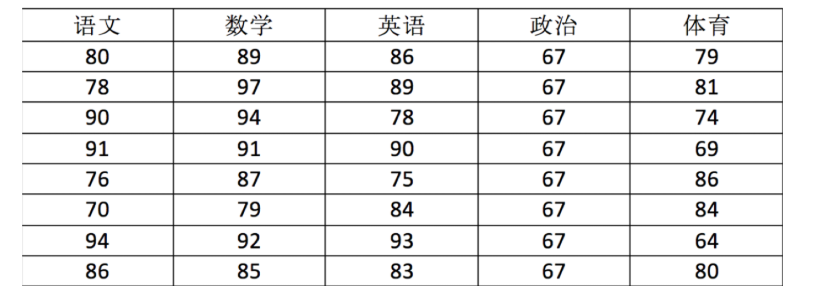用ndarray进行存储：
import numpy as np

# 创建ndarray
score = np.array(
[[80, 89, 86, 67, 79],
[78, 97, 89, 67, 81],
[90, 94, 78, 67, 74],
[91, 91, 90, 67, 69],
[76, 87, 75, 67, 86],
[70, 79, 84, 67, 84],
[94, 92, 93, 67, 64],
[86, 85, 83, 67, 80]])

score

返回结果：
array([[80, 89, 86, 67, 79],
[78, 97, 89, 67, 81],
[90, 94, 78, 67, 74],
[91, 91, 90, 67, 69],
[76, 87, 75, 67, 86],
[70, 79, 84, 67, 84],
[94, 92, 93, 67, 64],
[86, 85, 83, 67, 80]])

提问:
使用Python列表可以存储一维数组，通过列表的嵌套可以实现多维数组，那么为什么还需要使用Numpy的ndarray呢？
3 ndarray与Python原生list运算效率对比
在这里我们通过一段代码运行来体会到ndarray的好处
import random
import time
import numpy as np
a = []
for i in range(100000000):
a.append(random.random())

# 通过%time魔法方法, 查看当前行的代码运行一次所花费的时间
%time sum1=sum(a)

b=np.array(a)

%time sum2=np.sum(b)

其中第一个时间显示的是使用原生Python计算时间,第二个内容是使用numpy计算时间:
CPU times: user 852 ms, sys: 262 ms, total: 1.11 s
Wall time: 1.13 s
CPU times: user 133 ms, sys: 653 µs, total: 133 ms
Wall time: 134 ms

从中我们看到ndarray的计算速度要快很多，节约了时间。
机器学习的最大特点就是大量的数据运算，那么如果没有一个快速的解决方案，那可能现在python也在机器学习领域达不到好的效果。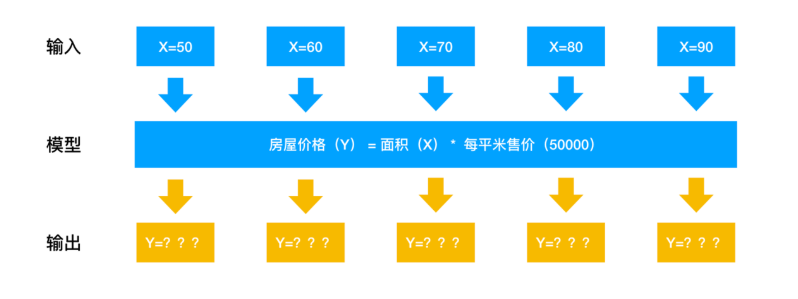Numpy专门针对ndarray的操作和运算进行了设计，所以数组的存储效率和输入输出性能远优于Python中的嵌套列表，数组越大，Numpy的优势就越明显。
思考：
ndarray为什么可以这么快？
4 ndarray的优势
4.1 内存块风格
ndarray到底跟原生python列表有什么不同呢，请看一张图：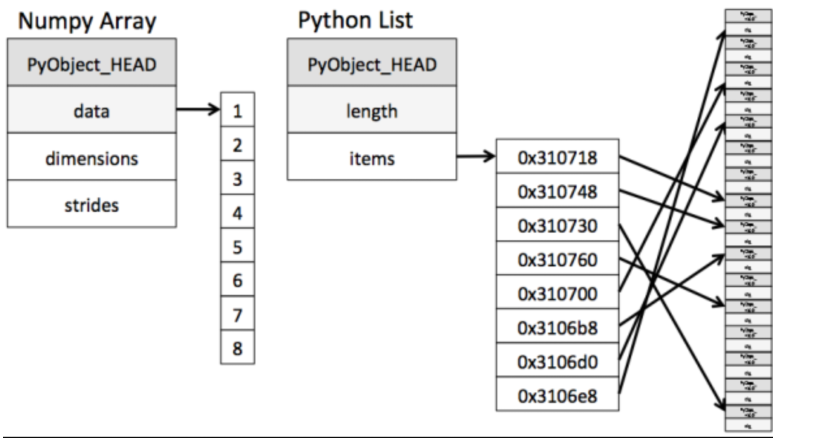从图中我们可以看出ndarray在存储数据的时候，数据与数据的地址都是连续的，这样就给使得批量操作数组元素时速度更快。
这是因为ndarray中的所有元素的类型都是相同的，而Python列表中的元素类型是任意的，所以ndarray在存储元素时内存可以连续，而python原生list就只能通过寻址方式找到下一个元素，这虽然也导致了在通用性能方面Numpy的ndarray不及Python原生list，但在科学计算中，Numpy的ndarray就可以省掉很多循环语句，代码使用方面比Python原生list简单的多。
4.2 ndarray支持并行化运算（向量化运算）
numpy内置了并行运算功能，当系统有多个核心时，做某种计算时，numpy会自动做并行计算
4.3 效率远高于纯Python代码
Numpy底层使用C语言编写，内部解除了GIL（全局解释器锁），其对数组的操作速度不受Python解释器的限制，所以，其效率远高于纯Python代码。
5 小结
numpy介绍【了解】
一个开源的Python科学计算库计算起来要比python简洁高效Numpy使用ndarray对象来处理多维数组ndarray介绍【了解】
NumPy提供了一个N维数组类型ndarray，它描述了相同类型的“items”的集合。生成numpy对象:np.array()ndarray的优势【掌握】
内存块风格
list -- 分离式存储,存储内容多样化ndarray -- 一体式存储,存储类型必须一样ndarray支持并行化运算（向量化运算）ndarray底层是用C语言写的,效率更高,释放了GIL

4.2 N维数组-ndarray
1 ndarray的属性
数组属性反映了数组本身固有的信息。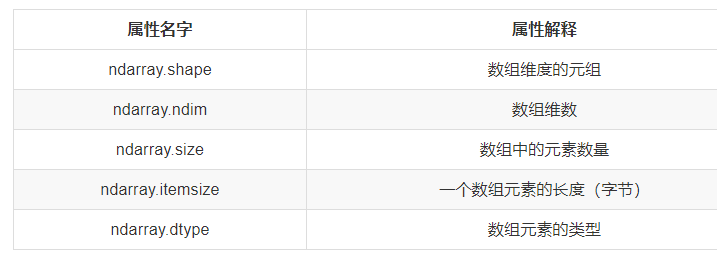2 ndarray的形状
首先创建一些数组。
# 创建不同形状的数组
>>> a = np.array([[1,2,3],[4,5,6]])
>>> b = np.array([1,2,3,4])
>>> c = np.array([[[1,2,3],[4,5,6]],[[1,2,3],[4,5,6]]])

分别打印出形状
>>> a.shape
>>> b.shape
>>> c.shape

(2, 3)  # 二维数组
(4,)    # 一维数组
(2, 2, 3) # 三维数组

如何理解数组的形状？
二维数组：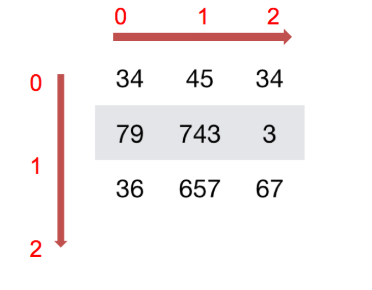三维数组：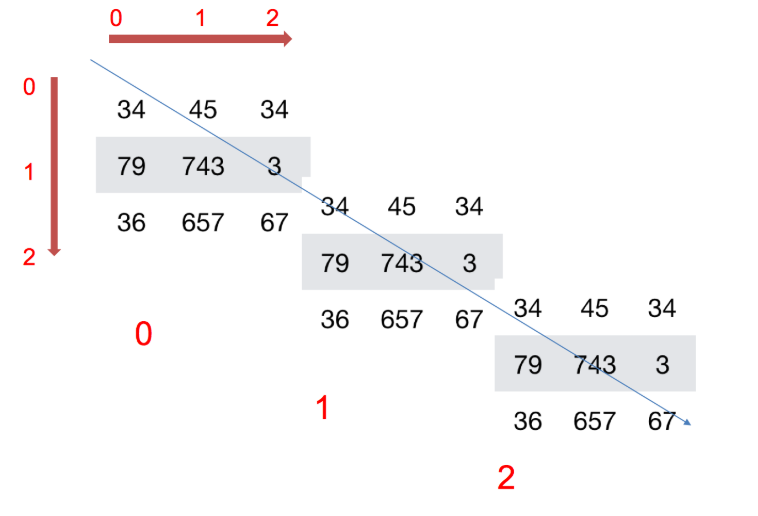3 ndarray的类型
>>> type(score.dtype)

<type 'numpy.dtype'>

dtype是numpy.dtype类型，先看看对于数组来说都有哪些类型
名称描述简写np.bool用一个字节存储的布尔类型（True或False）'b'np.int8一个字节大小，-128 至 127'i'np.int16整数，-32768 至 32767'i2'np.int32整数，-2^31​ 至 2^32 -1'i4'np.int64整数，-2^63 至 2^63 - 1'i8'np.uint8无符号整数，0 至 255'u'np.uint16无符号整数，0 至 65535'u2'np.uint32无符号整数，0 至 2^32 - 1'u4'np.uint64无符号整数，0 至 2^64 - 1'u8'np.float16半精度浮点数：16位，正负号1位，指数5位，精度10位'f2'np.float32单精度浮点数：32位，正负号1位，指数8位，精度23位'f4'np.float64双精度浮点数：64位，正负号1位，指数11位，精度52位'f8'np.complex64复数，分别用两个32位浮点数表示实部和虚部'c8'np.complex128复数，分别用两个64位浮点数表示实部和虚部'c16'np.object_python对象'O'np.string_字符串'S'np.unicode_unicode类型'U'
创建数组的时候指定类型
>>> a = np.array([[1, 2, 3],[4, 5, 6]], dtype=np.float32)
>>> a.dtype
dtype('float32')

>>> arr = np.array(['python', 'tensorflow', 'scikit-learn', 'numpy'], dtype = np.string_)
>>> arr
array([b'python', b'tensorflow', b'scikit-learn', b'numpy'], dtype='|S12')

注意：若不指定，整数默认int64，小数默认float64
4 总结
数组的基本属性【知道】
属性名字属性解释ndarray.shape数组维度的元组ndarray.ndim数组维数ndarray.size数组中的元素数量ndarray.itemsize一个数组元素的长度（字节）ndarray.dtype数组元素的类型

4.3 基本操作
1 生成数组的方法
1.1 生成0和1的数组
np.ones(shape, dtype)np.ones_like(a, dtype)np.zeros(shape, dtype)np.zeros_like(a, dtype)
ones = np.ones([4,8])
ones

返回结果:
array([[1., 1., 1., 1., 1., 1., 1., 1.],
[1., 1., 1., 1., 1., 1., 1., 1.],
[1., 1., 1., 1., 1., 1., 1., 1.],
[1., 1., 1., 1., 1., 1., 1., 1.]])

np.zeros_like(ones)

返回结果:
array([[0., 0., 0., 0., 0., 0., 0., 0.],
[0., 0., 0., 0., 0., 0., 0., 0.],
[0., 0., 0., 0., 0., 0., 0., 0.],
[0., 0., 0., 0., 0., 0., 0., 0.]])

1.2 从现有数组生成
1.2.1 生成方式
np.array(object, dtype)  np.asarray(a, dtype)
a = np.array([[1,2,3],[4,5,6]])
# 从现有的数组当中创建
a1 = np.array(a)
# 相当于索引的形式，并没有真正的创建一个新的
a2 = np.asarray(a)

1.2.2 关于array和asarray的不同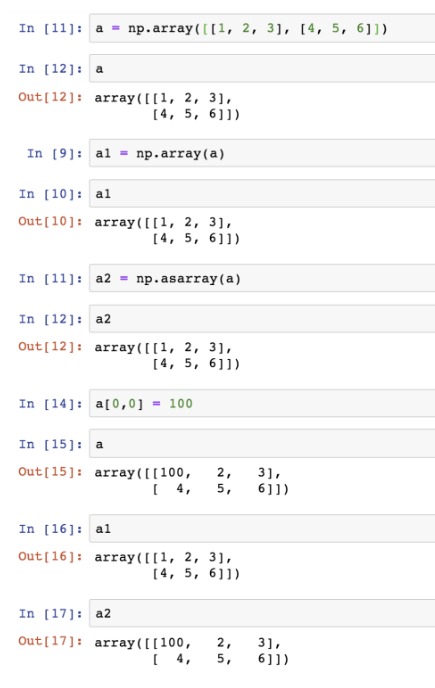1.3 生成固定范围的数组
1.3.1 np.linspace (start, stop, num, endpoint)
创建等差数组 — 指定数量参数:
start:序列的起始值stop:序列的终止值num:要生成的等间隔样例数量，默认为50endpoint:序列中是否包含stop值，默认为ture
# 生成等间隔的数组
np.linspace(0, 100, 11)

返回结果：
array([  0.,  10.,  20.,  30.,  40.,  50.,  60.,  70.,  80.,  90., 100.])

1.3.2 np.arange(start,stop, step, dtype)
创建等差数组 — 指定步长参数
step:步长,默认值为1
np.arange(10, 50, 2)

返回结果：
array([10, 12, 14, 16, 18, 20, 22, 24, 26, 28, 30, 32, 34, 36, 38, 40, 42,
44, 46, 48])

1.3.3 np.logspace(start,stop, num)
创建等比数列  参数:
num:要生成的等比数列数量，默认为50
# 生成10^x
np.logspace(0, 2, 3)

返回结果:
array([  1.,  10., 100.])

1.4 生成随机数组
1.4.1 使用模块介绍
np.random模块
1.4.2 正态分布
一、基础概念复习：正态分布（理解）
a. 什么是正态分布
正态分布是一种概率分布。正态分布是具有两个参数μ和σ的连续型随机变量的分布，第一参数μ是服从正态分布的随机变量的均值，第二个参数σ是此随机变量的标准差，所以正态分布记作N(μ，σ )。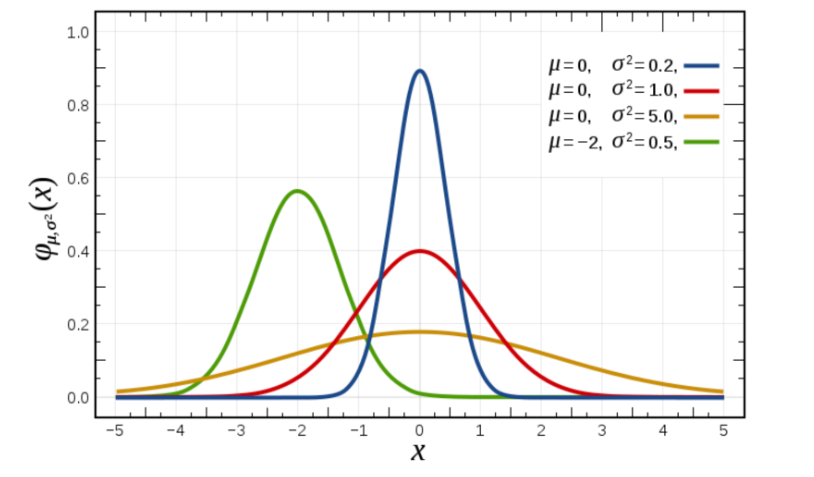b. 正态分布的应用
生活、生产与科学实验中很多随机变量的概率分布都可以近似地用正态分布来描述。
c. 正态分布特点
μ决定了其位置，其标准差σ决定了分布的幅度。当μ = 0,σ = 1时的正态分布是标准正态分布。
标准差如何来？
方差
是在概率论和统计方差衡量一组数据时离散程度的度量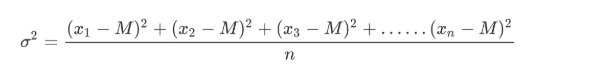其中M为平均值，n为数据总个数，σ 为标准差，σ ^2​可以理解一个整体为方差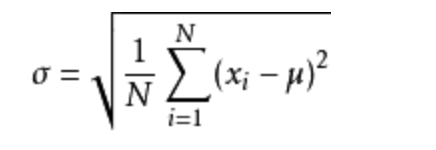标准差与方差的意义
可以理解成数据的一个离散程度的衡量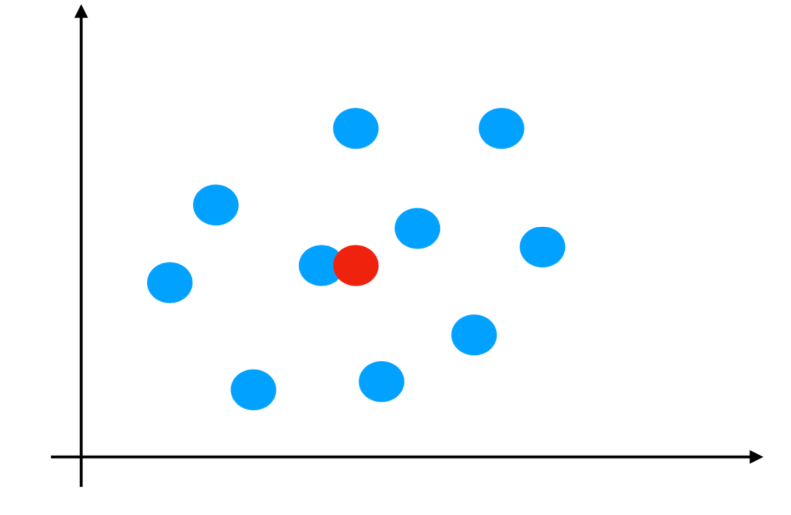二、正态分布创建方式
np.random.randn(d0, d1, …, dn) 功能：从标准正态分布中返回一个或多个样本值  np.random.normal(loc=0.0, scale=1.0, size=None) loc：float ​ 此概率分布的均值（对应着整个分布的中心centre） scale：float ​ 此概率分布的标准差（对应于分布的宽度，scale越大越矮胖，scale越小，越瘦高） size：int or tuple of ints ​ 输出的shape，默认为None，只输出一个值  np.random.standard_normal(size=None) 返回指定形状的标准正态分布的数组。
举例1：生成均值为1.75，标准差为1的正态分布数据，100000000个
x1 = np.random.normal(1.75, 1, 100000000)

返回结果：
array([2.90646763, 1.46737886, 2.21799024, ..., 1.56047411, 1.87969135,
0.9028096 ])

# 生成均匀分布的随机数
x1 = np.random.normal(1.75, 1, 100000000)

# 画图看分布状况
# 1）创建画布
plt.figure(figsize=(20, 10), dpi=100)

# 2）绘制直方图
plt.hist(x1, 1000)

# 3）显示图像
plt.show()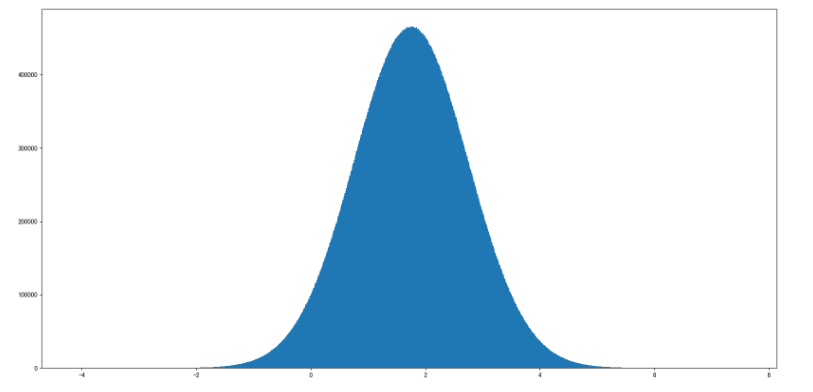例如：我们可以模拟生成一组股票的涨跌幅的数据
举例2：随机生成4支股票1周的交易日涨幅数据
4支股票，一周(5天)的涨跌幅数据，如何获取？
随机生成涨跌幅在某个正态分布内，比如均值0，方差1
股票涨跌幅数据的创建
# 创建符合正态分布的4只股票5天的涨跌幅数据
stock_change = np.random.normal(0, 1, (4, 5))
stock_change

返回结果：
array([[ 0.0476585 ,  0.32421568,  1.50062162,  0.48230497, -0.59998822],
[-1.92160851,  2.20430374, -0.56996263, -1.44236548,  0.0165062 ],
[-0.55710486, -0.18726488, -0.39972172,  0.08580347, -1.82842225],
[-1.22384505, -0.33199305,  0.23308845, -1.20473702, -0.31753223]])

1.4.2 均匀分布
np.random.rand(d0, d1, ..., dn)
返回[0.0，1.0)内的一组均匀分布的数。np.random.uniform(low=0.0, high=1.0, size=None)
功能：从一个均匀分布[low,high)中随机采样，注意定义域是左闭右开，即包含low，不包含high.参数介绍:
low: 采样下界，float类型，默认值为0；high: 采样上界，float类型，默认值为1；size: 输出样本数目，为int或元组(tuple)类型，例如，size=(m,n,k), 则输出mnk个样本，缺省时输出1个值。返回值：ndarray类型，其形状和参数size中描述一致。np.random.randint(low, high=None, size=None, dtype='l')
从一个均匀分布中随机采样，生成一个整数或N维整数数组，取数范围：若high不为None时，取[low,high)之间随机整数，否则取值[0,low)之间随机整数。
# 生成均匀分布的随机数
x2 = np.random.uniform(-1, 1, 100000000)

返回结果：
array([ 0.22411206,  0.31414671,  0.85655613, ..., -0.92972446,
0.95985223,  0.23197723])

画图看分布状况：
import matplotlib.pyplot as plt

# 生成均匀分布的随机数
x2 = np.random.uniform(-1, 1, 100000000)

# 画图看分布状况
# 1）创建画布
plt.figure(figsize=(10, 10), dpi=100)

# 2）绘制直方图
plt.hist(x=x2, bins=1000)  # x代表要使用的数据，bins表示要划分区间数

# 3）显示图像
plt.show()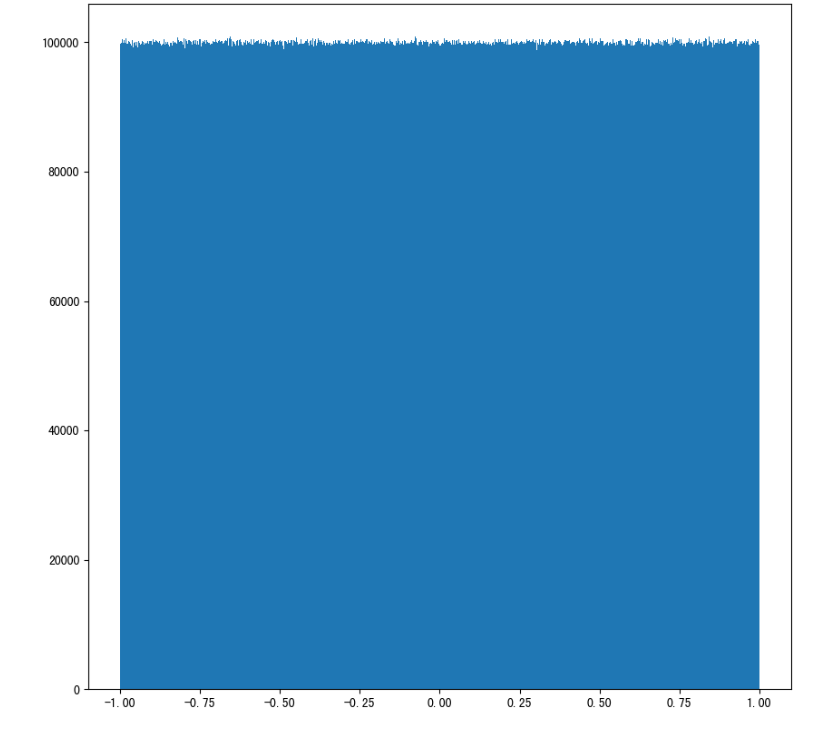2 数组的索引、切片
一维、二维、三维的数组如何索引？
直接进行索引,切片对象[:, :] -- 先行后列
二维数组索引方式：
举例：获取第一个股票的前3个交易日的涨跌幅数据
# 二维的数组，两个维度
stock_change[0, 0:3]

返回结果：
array([-0.03862668, -1.46128096, -0.75596237])

三维数组索引方式：
# 三维
a1 = np.array([ [[1,2,3],[4,5,6]], [[12,3,34],[5,6,7]]])
# 返回结果
array([[[ 1,  2,  3],
[ 4,  5,  6]],

[[12,  3, 34],
[ 5,  6,  7]]])
# 索引、切片
>>> a1[0, 0, 1]   # 输出: 2

3 形状修改
3.1 ndarray.reshape(shape, order)
返回一个具有相同数据域，但shape不一样的视图行、列不进行互换
# 在转换形状的时候，一定要注意数组的元素匹配
stock_change.reshape([5, 4])
stock_change.reshape([-1,10])  # 数组的形状被修改为: (2, 10), -1: 表示通过待计算

3.2 ndarray.resize(new_shape)
修改数组本身的形状（需要保持元素个数前后相同）行、列不进行互换
stock_change.resize([5, 4])

# 查看修改后结果
stock_change.shape
(5, 4)

3.3 ndarray.T
数组的转置将数组的行、列进行互换
stock_change.T.shape
(4, 5)

4 类型修改
4.1 ndarray.astype(type)
返回修改了类型之后的数组
stock_change.astype(np.int32)

4.2 ndarray.tostring([order])或者ndarray.tobytes([order])
构造包含数组中原始数据字节的Python字节
arr = np.array([[[1, 2, 3], [4, 5, 6]], [[12, 3, 34], [5, 6, 7]]])
arr.tostring()

4.3 jupyter输出太大可能导致崩溃问题【了解】
如果遇到
IOPub data rate exceeded.
The notebook server will temporarily stop sending output
to the client in order to avoid crashing it.
To change this limit, set the config variable
--NotebookApp.iopub_data_rate_limit.

这个问题是在jupyer当中对输出的字节数有限制，需要去修改配置文件
创建配置文件
jupyter notebook --generate-config
vi ~/.jupyter/jupyter_notebook_config.py

取消注释,多增加
## (bytes/sec) Maximum rate at which messages can be sent on iopub before they
#  are limited.
c.NotebookApp.iopub_data_rate_limit = 10000000

但是不建议这样去修改，jupyter输出太大会崩溃
5 数组的去重
5.1 np.unique()
temp = np.array([[1, 2, 3, 4],[3, 4, 5, 6]])
>>> np.unique(temp)
array([1, 2, 3, 4, 5, 6])

6 小结
创建数组【掌握】
生成0和1的数组
np.ones()np.ones_like()从现有数组中生成
np.array -- 深拷贝np.asarray -- 浅拷贝 生成固定范围数组
np.linspace()
nun -- 生成等间隔的多少个np.arange()
step -- 每间隔多少生成数据np.logspace()
生成以10的N次幂的数据 生层随机数组
正态分布
里面需要关注的参数:均值:u, 标准差:σ
u -- 决定了这个图形的左右位置σ -- 决定了这个图形是瘦高还是矮胖np.random.randn()np.random.normal(0, 1, 100)均匀
np.random.rand()np.random.uniform(0, 1, 100)np.random.randint(0, 10, 10) 数组索引【知道】
直接进行索引,切片对象[:, :] -- 先行后列 数组形状改变【掌握】
对象.reshape()
没有进行行列互换,新产生一个ndarray对象.resize()
没有进行行列互换,修改原来的ndarray对象.T
进行了行列互换 数组去重【知道】
np.unique(对象)

4.4 ndarray运算
问题
如果想要操作符合某一条件的数据，应该怎么做？
1 逻辑运算
# 生成10名同学，5门功课的数据
>>> score = np.random.randint(40, 100, (10, 5))

# 取出最后4名同学的成绩，用于逻辑判断
>>> test_score = score[6:, 0:5]

# 逻辑判断, 如果成绩大于60就标记为True 否则为False
>>> test_score > 60
array([[ True,  True,  True, False,  True],
[ True,  True,  True, False,  True],
[ True,  True, False, False,  True],
[False,  True,  True,  True,  True]])

# BOOL赋值, 将满足条件的设置为指定的值-布尔索引
>>> test_score[test_score > 60] = 1
>>> test_score
array([[ 1,  1,  1, 52,  1],
[ 1,  1,  1, 59,  1],
[ 1,  1, 44, 44,  1],
[59,  1,  1,  1,  1]])

2 通用判断函数
np.all()
# 判断前两名同学的成绩[0:2, :]是否全及格
>>> np.all(score[0:2, :] > 60)
False

np.any()
# 判断前两名同学的成绩[0:2, :]是否有大于90分的
>>> np.any(score[0:2, :] > 80)
True

3 np.where（三元运算符）
通过使用np.where能够进行更加复杂的运算
np.where()
# 判断前四名学生,前四门课程中，成绩中大于60的置为1，否则为0
temp = score[:4, :4]
np.where(temp > 60, 1, 0)

复合逻辑需要结合np.logical_and和np.logical_or使用
# 判断前四名学生,前四门课程中，成绩中大于60且小于90的换为1，否则为0
np.where(np.logical_and(temp > 60, temp < 90), 1, 0)

# 判断前四名学生,前四门课程中，成绩中大于90或小于60的换为1，否则为0
np.where(np.logical_or(temp > 90, temp < 60), 1, 0)

4 统计运算
如果想要知道学生成绩最大的分数，或者做小分数应该怎么做？
4.1 统计指标
在数据挖掘/机器学习领域，统计指标的值也是我们分析问题的一种方式。常用的指标如下：
min(a, axis)
Return the minimum of an array or minimum along an axis.max(a, axis])
Return the maximum of an array or maximum along an axis.median(a, axis)
Compute the median along the specified axis.mean(a, axis, dtype)
Compute the arithmetic mean along the specified axis.std(a, axis, dtype)
Compute the standard deviation along the specified axis.var(a, axis, dtype)
Compute the variance along the specified axis.
4.2 案例：学生成绩统计运算
进行统计的时候，axis 轴的取值并不一定，Numpy中不同的API轴的值都不一样，在这里，axis 0代表列, axis 1代表行去进行统计
# 接下来对于前四名学生,进行一些统计运算
# 指定列 去统计
temp = score[:4, 0:5]
print("前四名学生,各科成绩的最大分：{}".format(np.max(temp, axis=0)))
print("前四名学生,各科成绩的最小分：{}".format(np.min(temp, axis=0)))
print("前四名学生,各科成绩波动情况：{}".format(np.std(temp, axis=0)))
print("前四名学生,各科成绩的平均分：{}".format(np.mean(temp, axis=0)))

结果：
前四名学生,各科成绩的最大分：[96 97 72 98 89]
前四名学生,各科成绩的最小分：[55 57 45 76 77]
前四名学生,各科成绩波动情况：[16.25576821 14.92271758 10.40432602  8.0311892   4.32290412]
前四名学生,各科成绩的平均分：[78.5  75.75 62.5  85.   82.25]

如果需要统计出某科最高分对应的是哪个同学？
np.argmax(temp, axis=)np.argmin(temp, axis=)
print("前四名学生，各科成绩最高分对应的学生下标：{}".format(np.argmax(temp, axis=0)))

结果：
前四名学生，各科成绩最高分对应的学生下标：[0 2 0 0 1]

5 小结
逻辑运算【知道】
直接进行大于,小于的判断合适之后,可以直接进行赋值通用判断函数【知道】
np.all()np.any()统计运算【掌握】
np.max()np.min()np.median()np.mean()np.std()np.var()np.argmax(axis=) — 最大元素对应的下标np.argmin(axis=) — 最小元素对应的下标

4.5 数组间运算
1 数组与数的运算
arr = np.array([[1, 2, 3, 2, 1, 4], [5, 6, 1, 2, 3, 1]])
arr + 1
arr / 2

# 可以对比python列表的运算，看出区别
a = [1, 2, 3, 4, 5]
a * 3

2 数组与数组的运算
arr1 = np.array([[1, 2, 3, 2, 1, 4], [5, 6, 1, 2, 3, 1]])
arr2 = np.array([[1, 2, 3, 4], [3, 4, 5, 6]])

上面这个能进行运算吗，结果是不行的！
2.1 广播机制
数组在进行矢量化运算时，要求数组的形状是相等的。当形状不相等的数组执行算术运算的时候，就会出现广播机制，该机制会对数组进行扩展，使数组的shape属性值一样，这样，就可以进行矢量化运算了。下面通过一个例子进行说明：
arr1 = np.array([,,,])
arr1.shape
# (4, 1)

arr2 = np.array([1,2,3])
arr2.shape
# (3,)

arr1+arr2
# 结果是：
array([[1, 2, 3],
[2, 3, 4],
[3, 4, 5],
[4, 5, 6]])

上述代码中，数组arr1是4行1列，arr2是1行3列。这两个数组要进行相加，按照广播机制会对数组arr1和arr2都进行扩展，使得数组arr1和arr2都变成4行3列。
下面通过一张图来描述广播机制扩展数组的过程：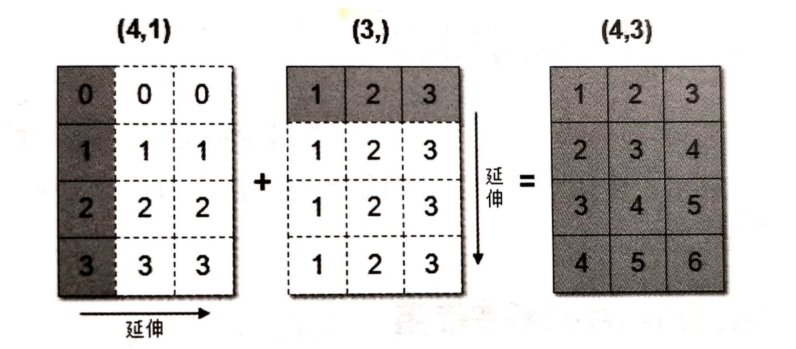这句话乃是理解广播的核心。广播主要发生在两种情况，一种是两个数组的维数不相等，但是它们的后缘维度的轴长相符，另外一种是有一方的长度为1。
广播机制实现了时两个或两个以上数组的运算，即使这些数组的shape不是完全相同的，只需要满足如下任意一个条件即可。
如果两个数组的后缘维度（trailing dimension，即从末尾开始算起的维度）的轴长度相符，或其中的一方的长度为1。
广播会在缺失和（或）长度为1的维度上进行。
广播机制需要扩展维度小的数组，使得它与维度最大的数组的shape值相同，以便使用元素级函数或者运算符进行运算。
如果是下面这样，则不匹配：
A  (1d array): 10
B  (1d array): 12
A  (2d array):      2 x 1
B  (3d array):  8 x 4 x 3

思考：下面两个ndarray是否能够进行运算？
arr1 = np.array([[1, 2, 3, 2, 1, 4], [5, 6, 1, 2, 3, 1]])
arr2 = np.array([, ])

3 小结
数组运算,满足广播机制,就OK【知道】
1.维度相等2.shape(其中对应的地方为1,也是可以的)

4.6 数学：矩阵
1 矩阵和向量
1.1 矩阵
矩阵，英文matrix，和array的区别矩阵必须是2维的，但是array可以是多维的。
如图:这个是 3×2 矩阵，即 3 行 2 列，如 m 为行，n 为列，那么 m×n 即 3×2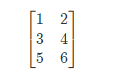矩阵的维数即行数×列数
矩阵元素(矩阵项):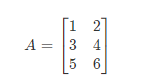Aij 指第 i 行，第 j 列的元素。
1.2 向量
向量是一种特殊的矩阵，讲义中的向量一般都是列向量，下面展示的就是三维列 向量(3×1)。)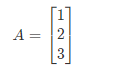2 加法和标量乘法
矩阵的加法:行列数相等的可以加。
例: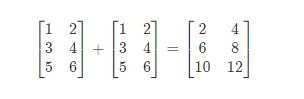矩阵的乘法:每个元素都要乘。
例: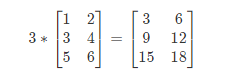组合算法也类似。
3 矩阵向量乘法
矩阵和向量的乘法如图：m×n 的矩阵乘以 n×1 的向量，得到的是 m×1 的向量
例: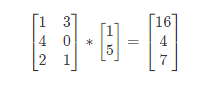1*1+3*5 = 16
4*1+0*5 = 4
2*1+1*5 = 7

矩阵乘法遵循准则：
(M行, N列)*(N行, L列) = (M行, L列)
4 矩阵乘法
矩阵乘法：
m×n 矩阵乘以 n×o 矩阵，变成 m×o 矩阵。
举例：比如说现在有两个矩阵 A 和 B，那 么它们的乘积就可以表示为图中所示的形式。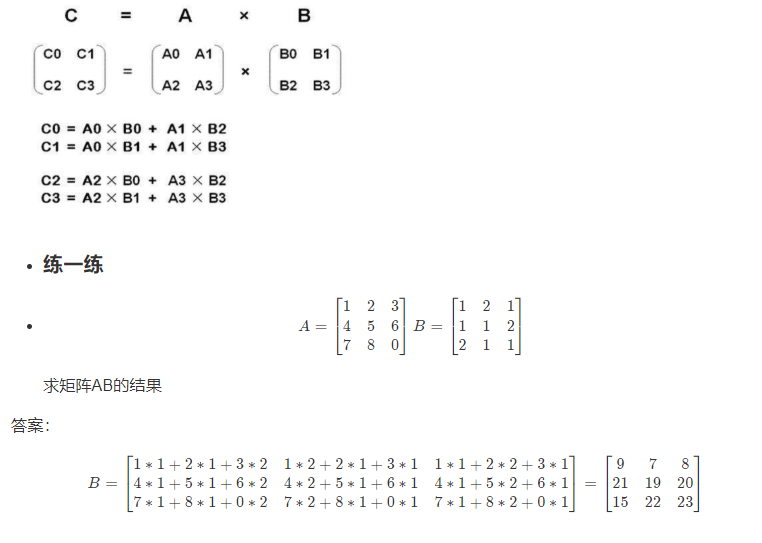5 矩阵乘法的性质
矩阵的乘法不满足交换律：A×B≠B×A
矩阵的乘法满足结合律。即：A×（B×C）=（A×B）×C
单位矩阵：在矩阵的乘法中，有一种矩阵起着特殊的作用，如同数的乘法中的 1,我们称 这种矩阵为单位矩阵．它是个方阵，一般用 I 或者 E 表示，从 左上角到右下角的对角线（称为主对角线）上的元素均为 1 以外全都为 0。如：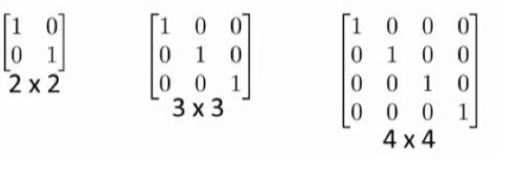6 逆、转置
矩阵的逆：如矩阵 A 是一个 m×m 矩阵（方阵），如果有逆矩阵，则：
AA-1 = A-1A = I
低阶矩阵求逆的方法:
​ 1.待定系数法
​ 2.初等变换
矩阵的转置：设 A 为 m×n 阶矩阵（即 m 行 n 列），第 i 行 j 列的元素是 a(i,j)，即：
A=a(i,j)
定义 A 的转置为这样一个 n×m 阶矩阵 B，满足 B=a(j,i)，即 b (i,j)=a (j,i)（B 的第 i 行第 j 列元素是 A 的第 j 行第 i 列元素），记 AT =B。
直观来看，将 A 的所有元素绕着一条从第 1 行第 1 列元素出发的右下方 45 度的射线作 镜面反转，即得到 A 的转置。
例：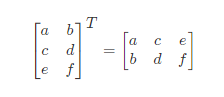7 矩阵运算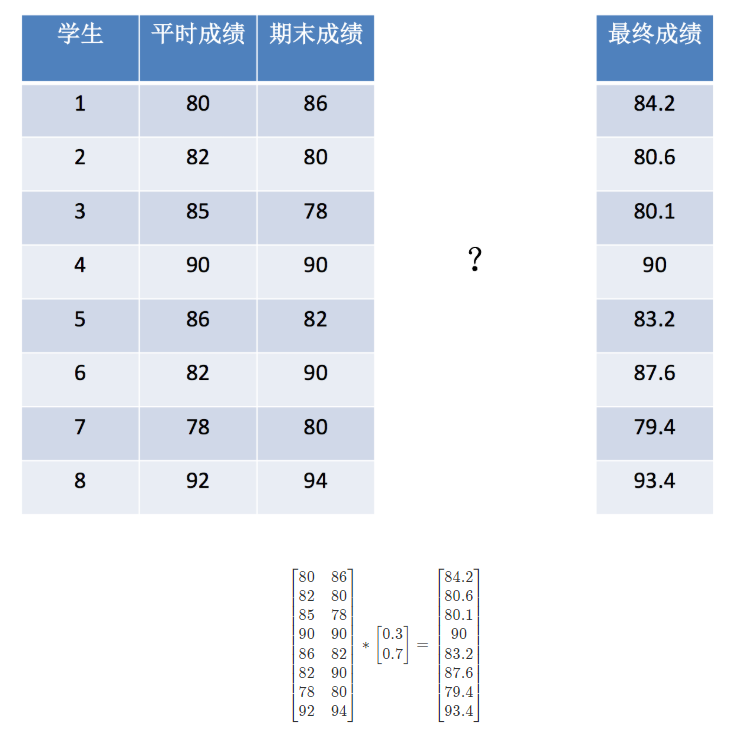7.1 矩阵乘法api：
np.matmulnp.dot
>>> a = np.array([[80, 86],
[82, 80],
[85, 78],
[90, 90],
[86, 82],
[82, 90],
[78, 80],
[92, 94]])
>>> b = np.array([[0.7], [0.3]])

>>> np.matmul(a, b)
array([[81.8],
[81.4],
[82.9],
[90. ],
[84.8],
[84.4],
[78.6],
[92.6]])

>>> np.dot(a,b)
array([[81.8],
[81.4],
[82.9],
[90. ],
[84.8],
[84.4],
[78.6],
[92.6]])

np.matmul和np.dot的区别:
二者都是矩阵乘法。 np.matmul中禁止矩阵与标量的乘法。 在矢量乘矢量的內积运算中，np.matmul与np.dot没有区别。
7 小结
1.矩阵和向量【知道】
矩阵就是特殊的二维数组向量就是一行或者一列的数据2.矩阵加法和标量乘法【知道】
矩阵的加法:行列数相等的可以加。矩阵的乘法:每个元素都要乘。3.矩阵和矩阵(向量)相乘 【知道】
(M行, N列)*(N行, L列) = (M行, L列)4.矩阵性质【知道】
矩阵不满足交换率,满足结合律5.单位矩阵【知道】
对角线都是1的矩阵,其他位置都为06.矩阵运算【掌握】
np.matmulnp.dot注意：二者都是矩阵乘法。 np.matmul中禁止矩阵与标量的乘法。 在矢量乘矢量的內积运算中，np.matmul与np.dot没有区别。

实现基础绘图功能
import matplotlib.pyplot as plt
import random
​
from pylab import mpl
# 设置显示中文字体
mpl.rcParams["font.sans-serif"] = ["SimHei"]
# 设置正常显示符号
mpl.rcParams["axes.unicode_minus"] = False
绘制基本图像
# 0.准备数据
x = range(60)
y_shanghai = [random.uniform(15, 18) for i in x]
​
# 1.创建画布
plt.figure(figsize=(20, 8), dpi=100)
​
# 2.绘制图像
plt.plot(x, y_shanghai)
​
# 3.图像显示
plt.show()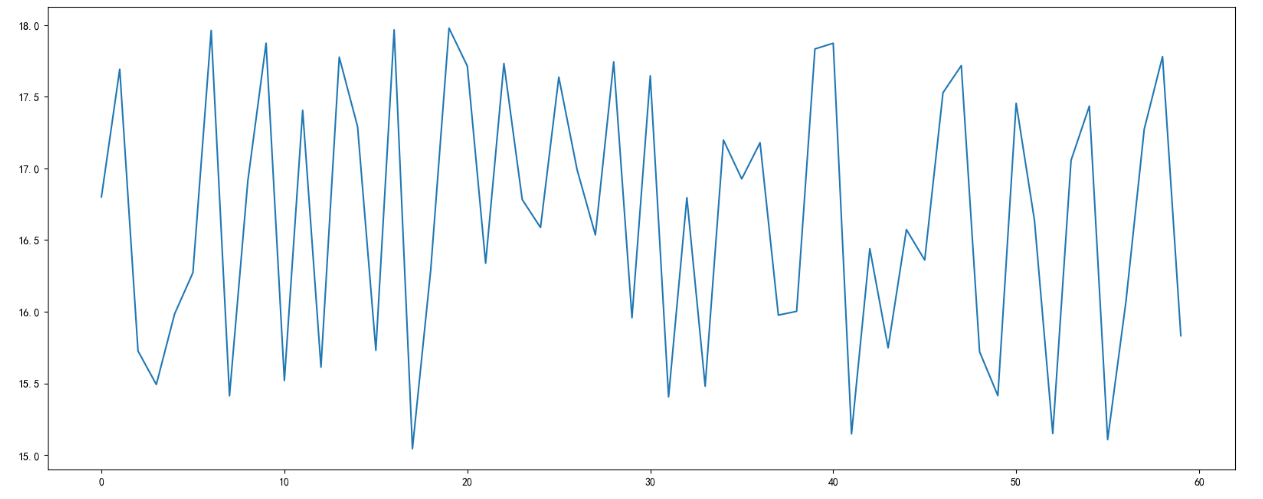# x,y
实现一些其他功能
# 0.准备数据
x = range(60)
y_shanghai = [random.uniform(15, 18) for i in x]
​
# 1.创建画布
plt.figure(figsize=(20, 8), dpi=100)
​
# 2.绘制图像
plt.plot(x, y_shanghai)
​
# 2.1 添加x,y轴刻度
# 设置x,y轴刻度
x_ticks_label = ["11点{}分".format(i) for i in x]
y_ticks = range(40)
​
# 修改x,y轴坐标刻度显示
# plt.xticks(x_ticks_label[::5]) # 坐标刻度不可以直接通过字符串进行修改
plt.xticks(x[::5], x_ticks_label[::5])
plt.yticks(y_ticks[::5])
​
# 2.2 添加网格显示
plt.grid(True, linestyle="--", alpha=1)
​
# 2.3 添加描述信息
plt.xlabel("时间")
plt.ylabel("温度")
plt.title("中午11点-12点某城市温度变化图", fontsize=20)
​
# 2.4 图像保存
plt.savefig("./test.png")
​
# 3.图像显示
plt.show()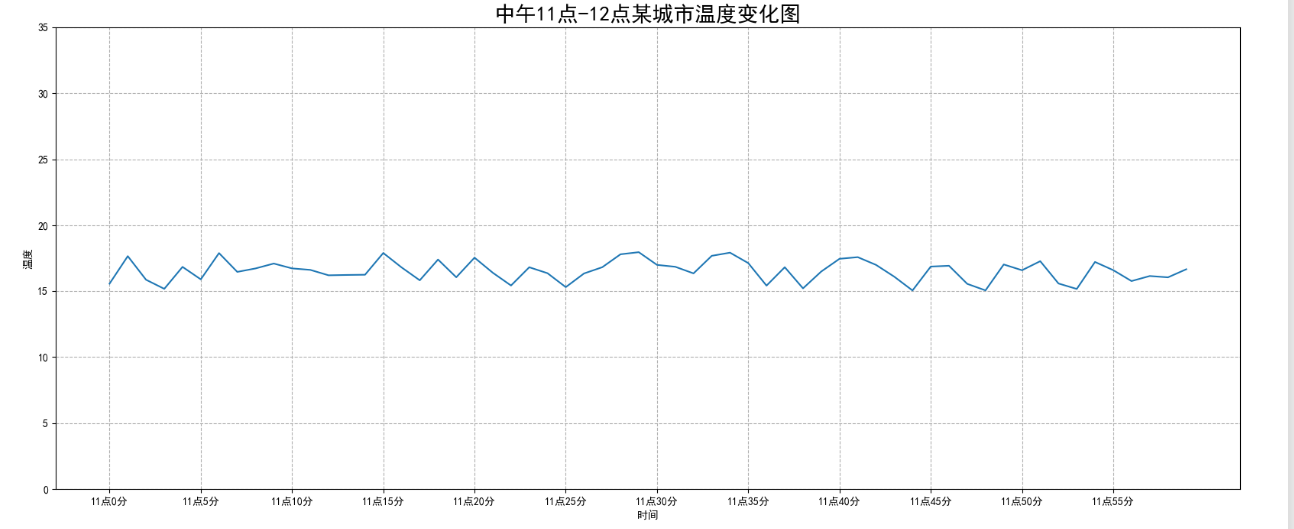# x_ticks_label
在一个坐标系中绘制多个图像
# 0.准备数据
x = range(60)
y_shanghai = [random.uniform(15, 18) for i in x]
y_beijing = [random.uniform(1, 3) for i in x]
​
# 1.创建画布
plt.figure(figsize=(20, 8), dpi=100)
​
# 2.绘制图像
plt.plot(x, y_shanghai, label="上海")
plt.plot(x, y_beijing, color="r", linestyle="--", label="北京")
​
# 2.1 添加x,y轴刻度
# 设置x,y轴刻度
x_ticks_label = ["11点{}分".format(i) for i in x]
y_ticks = range(40)
​
# 修改x,y轴坐标刻度显示
# plt.xticks(x_ticks_label[::5]) # 坐标刻度不可以直接通过字符串进行修改
#此处实际后者x_ticks_label[::5]会替换前者x[::5]，效果即只显示后者，前者不会显示即无效化
plt.xticks(x[::5], x_ticks_label[::5])
plt.yticks(y_ticks[::5])
​
# 2.2 添加网格显示
plt.grid(True, linestyle="--", alpha=1)
​
# 2.3 添加描述信息
plt.xlabel("时间")
plt.ylabel("温度")
plt.title("中午11点-12点某城市温度变化图", fontsize=20)
​
# 2.4 图像保存
plt.savefig("./test.png")
​
# 2.5 显示图例
plt.legend(loc=0)
​
# 3.图像显示
plt.show()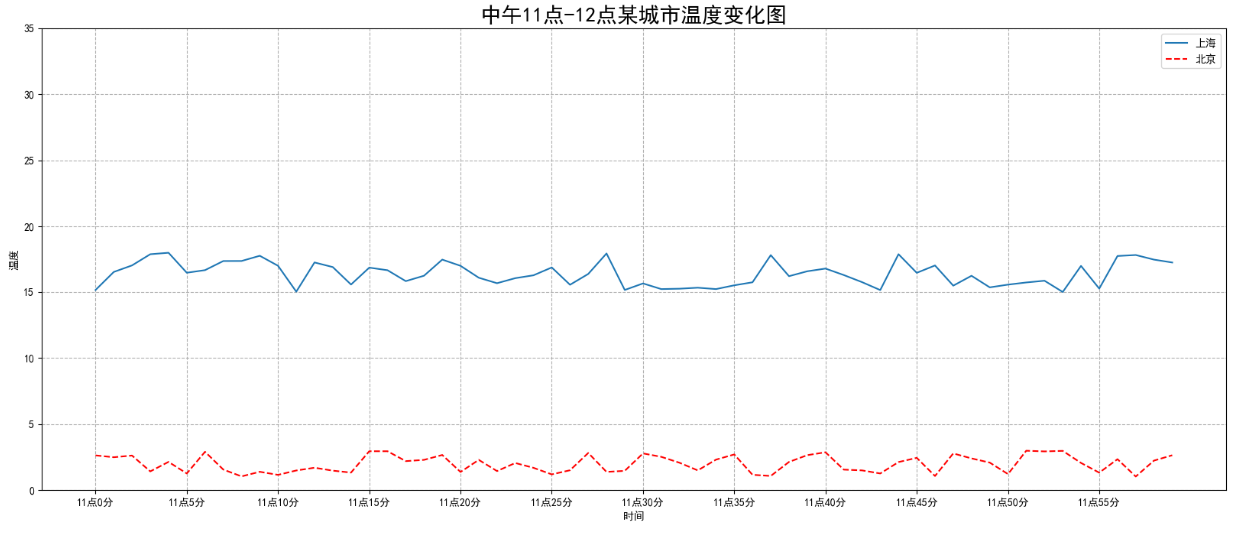多个坐标系实现绘图
# 0.准备数据
x = range(60)
y_shanghai = [random.uniform(15, 18) for i in x]
y_beijing = [random.uniform(1, 3) for i in x]
​
# 1.创建画布
# plt.figure(figsize=(20, 8), dpi=100)
fig, axes = plt.subplots(nrows=1, ncols=2, figsize=(20, 8), dpi=100)
​
# 2.绘制图像
# plt.plot(x, y_shanghai, label="上海")
# plt.plot(x, y_beijing, color="r", linestyle="--", label="北京")
axes.plot(x, y_shanghai, label="上海")
axes.plot(x, y_beijing, color="r", linestyle="--", label="北京")
​
# 2.1 添加x,y轴刻度
# 设置x,y轴刻度
x_ticks_label = ["11点{}分".format(i) for i in x]
y_ticks = range(40)
​
# 修改x,y轴坐标刻度显示
# plt.xticks(x_ticks_label[::5]) # 坐标刻度不可以直接通过字符串进行修改
# plt.xticks(x[::5], x_ticks_label[::5])
# plt.yticks(y_ticks[::5])
axes.set_xticks(x[::5])
axes.set_yticks(y_ticks[::5])
axes.set_xticklabels(x_ticks_label[::5])
axes.set_xticks(x[::5])
axes.set_yticks(y_ticks[::5])
axes.set_xticklabels(x_ticks_label[::5])
​
# 2.2 添加网格显示
# plt.grid(True, linestyle="--", alpha=1)
axes.grid(True, linestyle="--", alpha=1)
axes.grid(True, linestyle="--", alpha=1)
​
# 2.3 添加描述信息
# plt.xlabel("时间")
# plt.ylabel("温度")
# plt.title("中午11点-12点某城市温度变化图", fontsize=20)
axes.set_xlabel("时间")
axes.set_ylabel("温度")
axes.set_title("中午11点-12点某城市温度变化图", fontsize=20)
axes.set_xlabel("时间")
axes.set_ylabel("温度")
axes.set_title("中午11点-12点某城市温度变化图", fontsize=20)
​
# 2.4 图像保存
plt.savefig("./test.png")
​
# 2.5 显示图例
# plt.legend(loc=0)
axes.legend(loc=0)
axes.legend(loc=0)
​
# 3.图像显示
plt.show()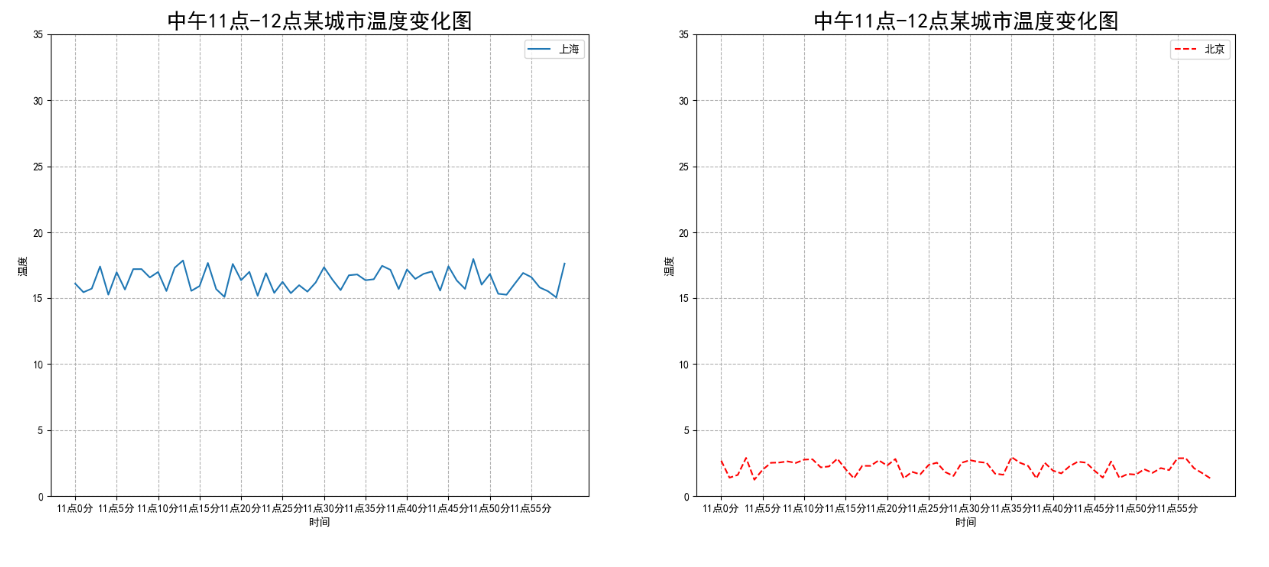折线图应用举例
import numpy as np
​
# 0.准备数据
x = np.linspace(-10, 10, 1000)
y = np.sin(x)
​
# 1.创建画布
plt.figure(figsize=(20, 8), dpi=100)
​
# 2.绘制函数图像
plt.plot(x, y)
​
# 2.1 添加网格显示
plt.grid()
​
# 3.显示图像
plt.show()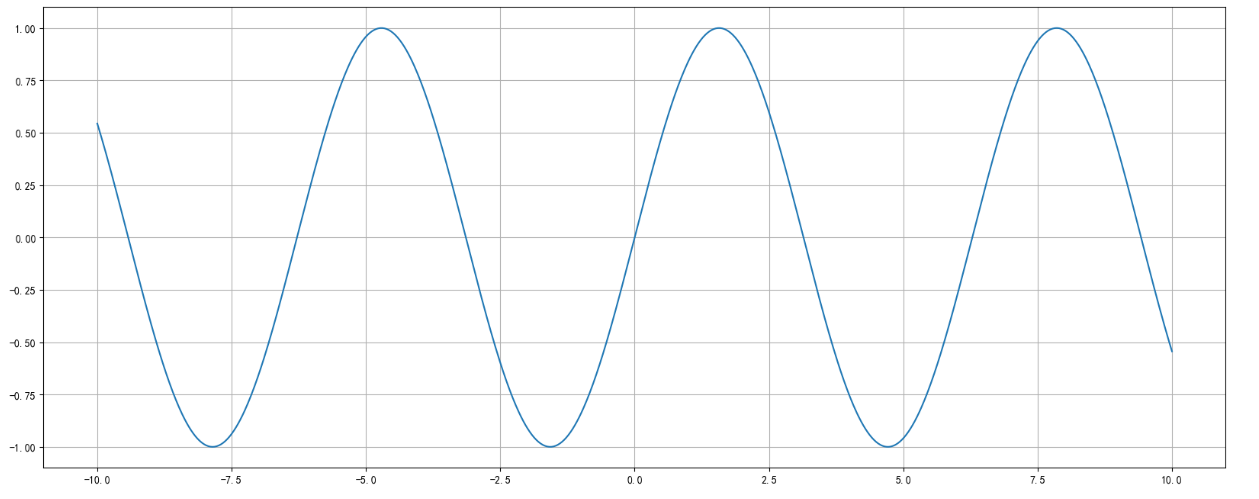import matplotlib.pyplot as plt

from pylab import mpl
# 设置显示中文字体
mpl.rcParams["font.sans-serif"] = ["SimHei"]
# 设置正常显示符号
mpl.rcParams["axes.unicode_minus"] = False
import matplotlib.pyplot as plt
​
from pylab import mpl
# 设置显示中文字体
mpl.rcParams["font.sans-serif"] = ["SimHei"]
# 设置正常显示符号
mpl.rcParams["axes.unicode_minus"] = False
散点图的绘制
# 0.准备数据
x = [225.98, 247.07, 253.14, 457.85, 241.58, 301.01,  20.67, 288.64,
163.56, 120.06, 207.83, 342.75, 147.9 ,  53.06, 224.72,  29.51,
21.61, 483.21, 245.25, 399.25, 343.35]
y = [196.63, 203.88, 210.75, 372.74, 202.41, 247.61,  24.9 , 239.34,
140.32, 104.15, 176.84, 288.23, 128.79,  49.64, 191.74,  33.1 ,
30.74, 400.02, 205.35, 330.64, 283.45]

# 1.创建画布
plt.figure(figsize=(20, 8), dpi=100)

# 2.绘制图像
plt.scatter(x, y)

# 3.图像显示
plt.show()
# 0.准备数据
x = [225.98, 247.07, 253.14, 457.85, 241.58, 301.01,  20.67, 288.64,
163.56, 120.06, 207.83, 342.75, 147.9 ,  53.06, 224.72,  29.51,
21.61, 483.21, 245.25, 399.25, 343.35]
y = [196.63, 203.88, 210.75, 372.74, 202.41, 247.61,  24.9 , 239.34,
140.32, 104.15, 176.84, 288.23, 128.79,  49.64, 191.74,  33.1 ,
30.74, 400.02, 205.35, 330.64, 283.45]
​
# 1.创建画布
plt.figure(figsize=(20, 8), dpi=100)
​
# 2.绘制图像
plt.scatter(x, y)
​
# 3.图像显示
plt.show()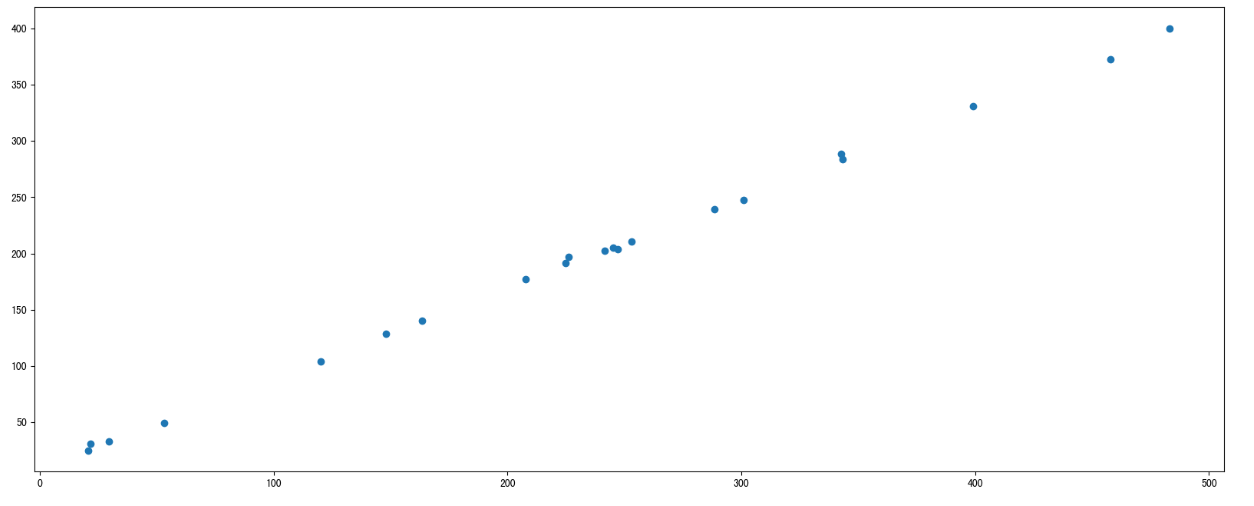柱状图绘制
# 0.准备数据
# 电影名字
movie_name = ['雷神3：诸神黄昏','正义联盟','东方快车谋杀案','寻梦环游记','全球风暴','降魔传','追捕','七十七天','密战','狂兽','其它']
# 横坐标
x = range(len(movie_name))
# 票房数据
y = [73853,57767,22354,15969,14839,8725,8716,8318,7916,6764,52222]
​
# 1.创建画布
plt.figure(figsize=(20, 8), dpi=100)
​
# 2.绘制图像
plt.bar(x, y, color=['b','r','g','y','c','m','y','k','c','g','b'], width=0.7)
​
# 2.1 修改x轴显示
plt.xticks(x, movie_name)
​
# 2.2 添加网格
plt.grid(linestyle="--", alpha=0.8)
​
# 2.3 添加标题
plt.title("电影票房收入对比")
​
# 3.图像显示
plt.show()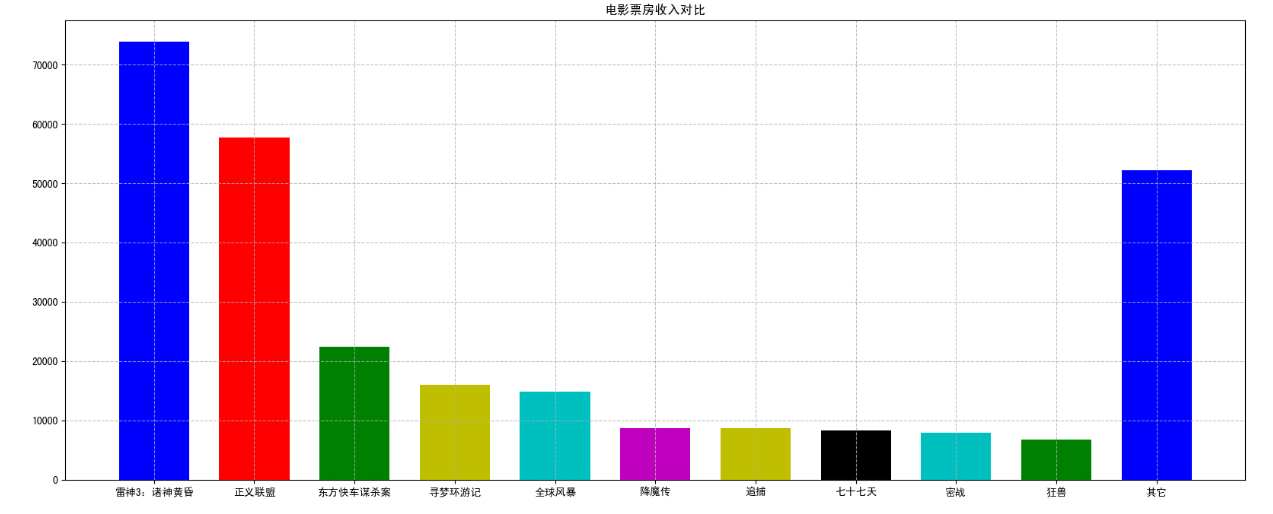import numpy as np
hello-numpy
初始numpy
score = np.array(
[[80, 89, 86, 67, 79],
[78, 97, 89, 67, 81],
[90, 94, 78, 67, 74],
[91, 91, 90, 67, 69],
[76, 87, 75, 67, 86],
[70, 79, 84, 67, 84],
[94, 92, 93, 67, 64],
[86, 85, 83, 67, 80]]
)
score
array([[80, 89, 86, 67, 79],
[78, 97, 89, 67, 81],
[90, 94, 78, 67, 74],
[91, 91, 90, 67, 69],
[76, 87, 75, 67, 86],
[70, 79, 84, 67, 84],
[94, 92, 93, 67, 64],
[86, 85, 83, 67, 80]])
ndarray和原生Python效率对比
import random
import time
import numpy as np
a = []
for i in range(100000000):
a.append(random.random())
​
# 通过%time魔法方法, 查看当前行的代码运行一次所花费的时间
%time sum1=sum(a)
​
b=np.array(a)
​
%time sum2=np.sum(b)
ndarray的使用
数组的属性
score
array([[80, 89, 86, 67, 79],
[78, 97, 89, 67, 81],
[90, 94, 78, 67, 74],
[91, 91, 90, 67, 69],
[76, 87, 75, 67, 86],
[70, 79, 84, 67, 84],
[94, 92, 93, 67, 64],
[86, 85, 83, 67, 80]])
score.shape
(8, 5)
score.ndim
2
score.size
40
score.itemsize
8
score.dtype
dtype('int64')
数组的形状
a = np.array([1,2,3])
a
array([1, 2, 3])
a.shape
(3,)
b = np.array([[1, 2, 3], [4, 5, 6]])
b
array([[1, 2, 3],
[4, 5, 6]])
b.shape
(2, 3)
c = np.array([[[1, 2, 3], [4, 5, 6]], [[1, 2, 3], [4, 5, 6]]])
c
array([[[1, 2, 3],
[4, 5, 6]],

[[1, 2, 3],
[4, 5, 6]]])
c.shape
(2, 2, 3)
数组的类型
b = np.array([[1, 2, 3], [4, 5, 6]], dtype=np.float32)
b
array([[1., 2., 3.],
[4., 5., 6.]], dtype=float32)
arr = np.array(["pythonI", "hello", "I"], dtype=np.string_)
arr
array([b'pythonI', b'hello', b'I'], dtype='|S7')

import numpy as np
import matplotlib.pyplot as plt
生成数组的方法
生成0,1 数组
ones = np.ones([4,8])
ones
array([[1., 1., 1., 1., 1., 1., 1., 1.],
[1., 1., 1., 1., 1., 1., 1., 1.],
[1., 1., 1., 1., 1., 1., 1., 1.],
[1., 1., 1., 1., 1., 1., 1., 1.]])
np.zeros_like(ones)
array([[0., 0., 0., 0., 0., 0., 0., 0.],
[0., 0., 0., 0., 0., 0., 0., 0.],
[0., 0., 0., 0., 0., 0., 0., 0.],
[0., 0., 0., 0., 0., 0., 0., 0.]])
从现在数组中生成
a = np.array([[1, 2, 3], [4, 5, 6]])
a
array([[1, 2, 3],
[4, 5, 6]])
a1 = np.array(a) # 深拷贝
a1
array([[1, 2, 3],
[4, 5, 6]])
a2 = np.asarray(a) # 浅拷贝
a2
array([[1, 2, 3],
[4, 5, 6]])
a[0, 0] = 1000
a
array([[1000,    2,    3],
[   4,    5,    6]])
a1
array([[1, 2, 3],
[4, 5, 6]])
a2
array([[1000,    2,    3],
[   4,    5,    6]])
生成固定范围的数组
np.linspace(0, 100, 11)
array([  0.,  10.,  20.,  30.,  40.,  50.,  60.,  70.,  80.,  90., 100.])
np.arange(10, 100, 2)
array([10, 12, 14, 16, 18, 20, 22, 24, 26, 28, 30, 32, 34, 36, 38, 40, 42,
44, 46, 48, 50, 52, 54, 56, 58, 60, 62, 64, 66, 68, 70, 72, 74, 76,
78, 80, 82, 84, 86, 88, 90, 92, 94, 96, 98])
np.logspace(0, 2, 3)
array([  1.,  10., 100.])
生成随机数组
正态分布
x1 = np.random.normal(1.75, 1, 100000000)
x1
array([2.14726132, 2.34220066, 1.24955806, ..., 0.27842733, 0.90682495,
1.75303785])
# 1.创建画布
plt.figure(figsize=(20, 8), dpi=100)
​
# 2.绘制图像
plt.hist(x1, 1000)
​
# 3.显示图像
plt.show()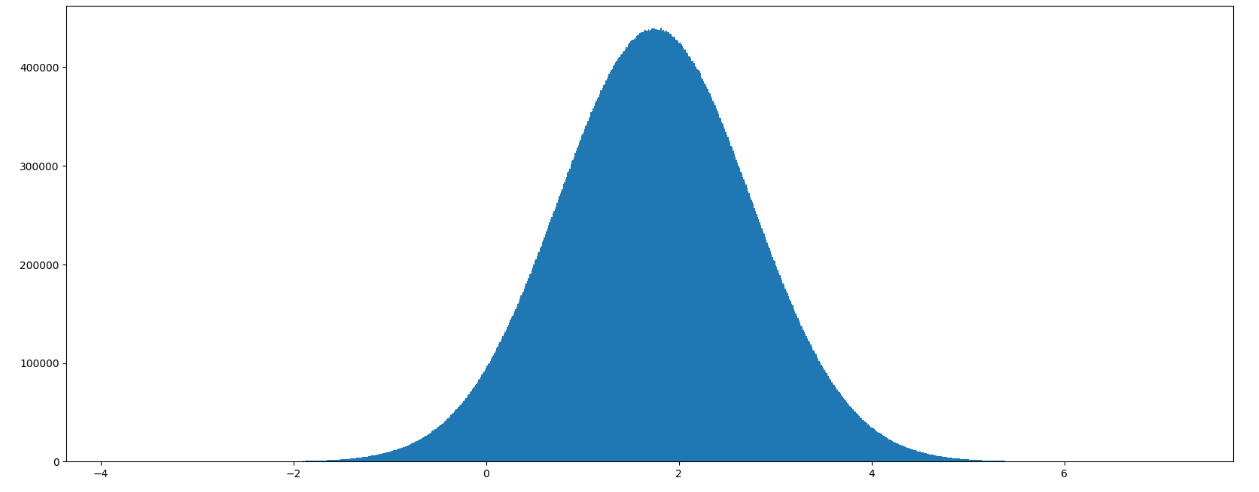stock_change = np.random.normal(0, 1, [4,5])
stock_change
均匀分布
x2 = np.random.uniform(-1, 1, 100000000)
x2
array([-0.2977719 , -0.10336683, -0.77723208, ...,  0.85248355,
-0.52078581,  0.79849061])
# 1.创建画布
plt.figure(figsize=(20, 8), dpi=100)
​
# 2.绘制图像
plt.hist(x2, 1000)
​
# 3.显示图像
plt.show()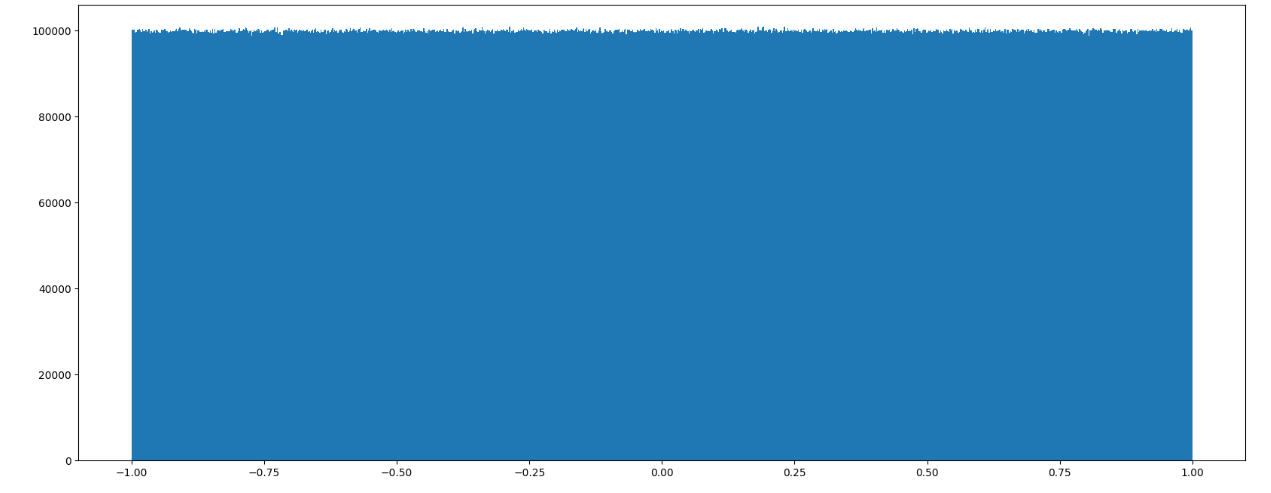数组的索引、切片
stock_change
array([[-0.5142373 , -0.32586912,  0.39132714,  1.02290317, -0.33438889],
[-0.08775205,  1.99655647,  0.24488145, -1.25742494, -0.35522986],
[ 0.85280747,  1.87762957,  0.68582294,  1.05605474, -1.6015672 ],
[-0.53759709,  1.62663522, -0.2319302 , -0.27205088, -0.49244907]])
stock_change[0, 0:3]
array([-0.5142373 , -0.32586912,  0.39132714])
a1 = np.array([ [[1,2,3],[4,5,6]], [[12,3,34],[5,6,7]]])
a1
array([[[ 1,  2,  3],
[ 4,  5,  6]],

[[12,  3, 34],
[ 5,  6,  7]]])
a1[1,0,0]
12
形状修改
stock_change.shape
(4, 5)
stock_change
array([[-0.5142373 , -0.32586912,  0.39132714,  1.02290317, -0.33438889],
[-0.08775205,  1.99655647,  0.24488145, -1.25742494, -0.35522986],
[ 0.85280747,  1.87762957,  0.68582294,  1.05605474, -1.6015672 ],
[-0.53759709,  1.62663522, -0.2319302 , -0.27205088, -0.49244907]])
stock_change.reshape([5,4])
array([[-0.5142373 , -0.32586912,  0.39132714,  1.02290317],
[-0.33438889, -0.08775205,  1.99655647,  0.24488145],
[-1.25742494, -0.35522986,  0.85280747,  1.87762957],
[ 0.68582294,  1.05605474, -1.6015672 , -0.53759709],
[ 1.62663522, -0.2319302 , -0.27205088, -0.49244907]])
stock_change.reshape([-1, 2])
array([[-0.5142373 , -0.32586912],
[ 0.39132714,  1.02290317],
[-0.33438889, -0.08775205],
[ 1.99655647,  0.24488145],
[-1.25742494, -0.35522986],
[ 0.85280747,  1.87762957],
[ 0.68582294,  1.05605474],
[-1.6015672 , -0.53759709],
[ 1.62663522, -0.2319302 ],
[-0.27205088, -0.49244907]])
# stock_change.reshape([3,-1]) # 报错
stock_change
array([[-0.5142373 , -0.32586912,  0.39132714,  1.02290317, -0.33438889],
[-0.08775205,  1.99655647,  0.24488145, -1.25742494, -0.35522986],
[ 0.85280747,  1.87762957,  0.68582294,  1.05605474, -1.6015672 ],
[-0.53759709,  1.62663522, -0.2319302 , -0.27205088, -0.49244907]])
stock_change.resize([10, 2])
stock_change
array([[-0.5142373 , -0.32586912],
[ 0.39132714,  1.02290317],
[-0.33438889, -0.08775205],
[ 1.99655647,  0.24488145],
[-1.25742494, -0.35522986],
[ 0.85280747,  1.87762957],
[ 0.68582294,  1.05605474],
[-1.6015672 , -0.53759709],
[ 1.62663522, -0.2319302 ],
[-0.27205088, -0.49244907]])
stock_change.T
array([[-0.5142373 ,  0.39132714, -0.33438889,  1.99655647, -1.25742494,
0.85280747,  0.68582294, -1.6015672 ,  1.62663522, -0.27205088],
[-0.32586912,  1.02290317, -0.08775205,  0.24488145, -0.35522986,
1.87762957,  1.05605474, -0.53759709, -0.2319302 , -0.49244907]])
类型修改
stock_change.astype(np.int64)
array([[ 0,  0],
[ 0,  1],
[ 0,  0],
[ 1,  0],
[-1,  0],
[ 0,  1],
[ 0,  1],
[-1,  0],
[ 1,  0],
[ 0,  0]])
a1 = np.array([ [[1,2,3],[4,5,6]], [[12,3,34],[5,6,7]]])
a1
array([[[ 1,  2,  3],
[ 4,  5,  6]],

[[12,  3, 34],
[ 5,  6,  7]]])
a1.tostring()
b'\x01\x00\x00\x00\x00\x00\x00\x00\x02\x00\x00\x00\x00\x00\x00\x00\x03\x00\x00\x00\x00\x00\x00\x00\x04\x00\x00\x00\x00\x00\x00\x00\x05\x00\x00\x00\x00\x00\x00\x00\x06\x00\x00\x00\x00\x00\x00\x00\x0c\x00\x00\x00\x00\x00\x00\x00\x03\x00\x00\x00\x00\x00\x00\x00"\x00\x00\x00\x00\x00\x00\x00\x05\x00\x00\x00\x00\x00\x00\x00\x06\x00\x00\x00\x00\x00\x00\x00\x07\x00\x00\x00\x00\x00\x00\x00'
数组的去重
a = np.array([[1,2,3,4],[2,3,4,5]])
a
array([[1, 2, 3, 4],
[2, 3, 4, 5]])
np.unique(a)
array([1, 2, 3, 4, 5])


import numpy as np
逻辑运算
score = np.random.randint(40, 100, (10, 5))
score
array([[89, 56, 51, 79, 91],
[66, 95, 57, 50, 58],
[44, 88, 79, 83, 74],
[76, 71, 44, 65, 44],
[96, 45, 45, 91, 76],
[53, 52, 54, 90, 70],
[97, 50, 73, 48, 50],
[90, 46, 60, 71, 44],
[94, 40, 52, 41, 73],
[96, 95, 68, 69, 47]])
test_score = score[6:, 0:5]
test_score
array([[97, 50, 73, 48, 50],
[90, 46, 60, 71, 44],
[94, 40, 52, 41, 73],
[96, 95, 68, 69, 47]])
test_score > 60
array([[ True, False,  True, False, False],
[ True, False, False,  True, False],
[ True, False, False, False,  True],
[ True,  True,  True,  True, False]])
test_score[test_score > 60] = 1
test_score
array([[ 1, 50,  1, 48, 50],
[ 1, 46, 60,  1, 44],
[ 1, 40, 52, 41,  1],
[ 1,  1,  1,  1, 47]])
通用判断函数
np.all(score[0:2, :] > 60)
False
score
array([[89, 56, 51, 79, 91],
[66, 95, 57, 50, 58],
[44, 88, 79, 83, 74],
[76, 71, 44, 65, 44],
[96, 45, 45, 91, 76],
[53, 52, 54, 90, 70],
[ 1, 50,  1, 48, 50],
[ 1, 46, 60,  1, 44],
[ 1, 40, 52, 41,  1],
[ 1,  1,  1,  1, 47]])
np.any(score[0:2, :] > 90)
True
np.where（三元运算符）
temp = score[:4, :4]
temp
array([[89, 56, 51, 79],
[66, 95, 57, 50],
[44, 88, 79, 83],
[76, 71, 44, 65]])
np.where(temp > 60, 1, 0)
array([[1, 0, 0, 1],
[1, 1, 0, 0],
[0, 1, 1, 1],
[1, 1, 0, 1]])
np.where(np.logical_and(temp > 60, temp < 90), 1, 0)
array([[1, 0, 0, 1],
[1, 0, 0, 0],
[0, 1, 1, 1],
[1, 1, 0, 1]])
np.where(np.logical_or(temp > 90, temp < 60), 1, 0)
array([[0, 1, 1, 0],
[0, 1, 1, 1],
[1, 0, 0, 0],
[0, 0, 1, 0]])
统计运算
temp = score[:4, :]
temp
array([[89, 56, 51, 79, 91],
[66, 95, 57, 50, 58],
[44, 88, 79, 83, 74],
[76, 71, 44, 65, 44]])
np.max(temp)
95
np.mean(temp)
68.0
np.max(temp, axis=0)
array([89, 95, 79, 83, 91])
np.max(temp, axis=1)
array([91, 95, 88, 76])
np.argmax(temp)
6
np.argmax(temp, axis=0)
array([0, 1, 2, 2, 0])
np.argmin(temp, axis=0)
array([2, 0, 3, 1, 3])
数组间的运算
a = np.array([[1,2,3],[3,4,5]])
a
array([[1, 2, 3],
[3, 4, 5]])
a + 3
array([[4, 5, 6],
[6, 7, 8]])
a / 2
array([[0.5, 1. , 1.5],
[1.5, 2. , 2.5]])
a = [1,2,3]
a * 3
[1, 2, 3, 1, 2, 3, 1, 2, 3]
arr1 = np.array([[1, 2, 3, 2, 1, 4], [5, 6, 1, 2, 3, 1]])
arr2 = np.array([[1, 2, 3, 4], [3, 4, 5, 6]])
# arr1 + arr2 # 不可以进行计算
arr1 = np.array([[1, 2, 3, 2, 1, 4], [5, 6, 1, 2, 3, 1]])
arr2 = np.array([, ])
arr1.shape
(2, 6)
arr2.shape
(2, 1)
arr2+arr1
array([[2, 3, 4, 3, 2, 5],
[8, 9, 4, 5, 6, 4]])
矩阵运算
a = np.array([[80, 86],
[82, 80],
[85, 78],
[90, 90],
[86, 82],
[82, 90],
[78, 80],
[92, 94]])
a
array([[80, 86],
[82, 80],
[85, 78],
[90, 90],
[86, 82],
[82, 90],
[78, 80],
[92, 94]])
b = np.array([[0.7], [0.3]])
b
array([[0.7],
[0.3]])
np.dot(a,b)
array([[81.8],
[81.4],
[82.9],
[90. ],
[84.8],
[84.4],
[78.6],
[92.6]])
np.matmul(a,b)
array([[81.8],
[81.4],
[82.9],
[90. ],
[84.8],
[84.4],
[78.6],
[92.6]])
c = 10
np.dot(c, a)
array([[800, 860],
[820, 800],
[850, 780],
[900, 900],
[860, 820],
[820, 900],
[780, 800],
[920, 940]])
# np.matmul(c, a)  # matmul不支持矩阵和标量的乘法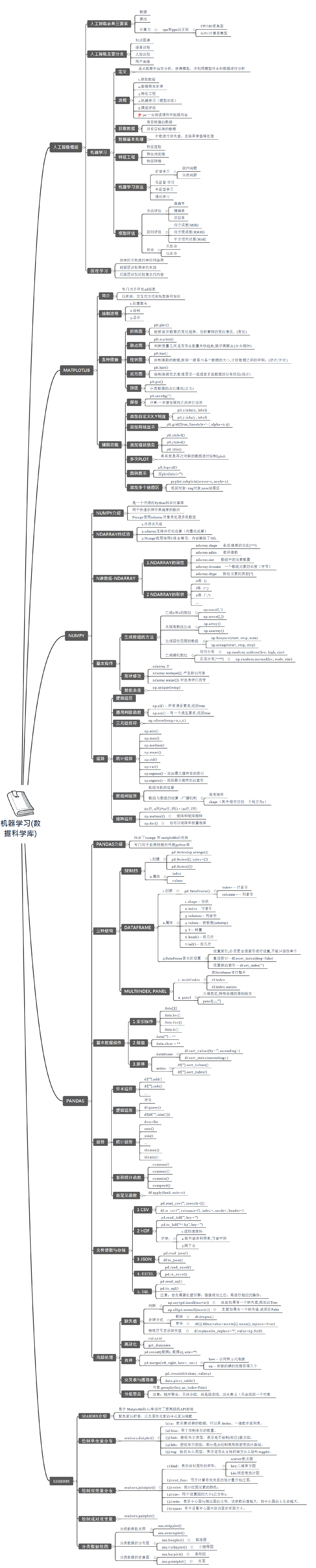展开全文• ## numpy

2020-02-09 09:11:18
如果你使用 Python 语言进行科学计算，那么一定会接触到 NumPyNumPy 是支持 Python 语言的数值计算扩充库，其拥有强大的多维数组处理与矩阵运算能力。除此之外，NumPy 还内建了大量的函数，方便你快速构建数学模型...
介绍 如果你使用 Python 语言进行科学计算，那么一定会接触到 NumPy。NumPy 是支持 Python 语言的数值计算扩充库，其拥有强大的多维数组处理与矩阵运算能力。除此之外，NumPy 还内建了大量的函数，方便你快速构建数学模型。
知识点 数值类型及多维数组 数组操作及随机抽样 数学函数及代数运算 数组索引及其他用法
NumPy 的英文全称为 Numerical Python，意味 Python 面向数值计算的第三方库。NumPy 的特点在于，针对 Python 内建的数组类型做了扩充，支持更高维度的数组和矩阵运算，以及更丰富的数学函数。
NumPy 是 Scipy.org 中最重要的库之一，它同时也被 Pandas，Matplotlib 等我们熟知的第三方库作为核心计算库。当你在单独安装这些库时，你会发现同时会安装 NumPy 作为依赖。
NumPy 数组类型 我们先来了解 NumPy 支持的数据类型。Python 本身支持的数值类型有 int（整型，Python 2 中存在 long 长整型）、float（浮点型）、bool（布尔型） 和 complex（复数型）。
而 NumPy 支持比 Python 本身更为丰富的数值类型，细分如下：
类型 解释 bool 布尔类型，1 个字节，值为 True 或 False。 int 整数类型，通常为 int64 或 int32 。 intc 与 C 里的 int 相同，通常为 int32 或 int64。 intp 用于索引，通常为 int32 或 int64。 int8 字节（从 -128 到 127） int16 整数（从 -32768 到 32767） int32 整数（从 -2147483648 到 2147483647） int64 整数（从 -9223372036854775808 到 9223372036854775807） uint8 无符号整数（从 0 到 255） uint16 无符号整数（从 0 到 65535） uint32 无符号整数（从 0 到 4294967295） uint64 无符号整数（从 0 到 18446744073709551615） float float64 的简写。 float16 半精度浮点，5 位指数，10 位尾数 float32 单精度浮点，8 位指数，23 位尾数 float64 双精度浮点，11 位指数，52 位尾数 complex complex128 的简写。 complex64 复数，由两个 32 位浮点表示。 complex128 复数，由两个 64 位浮点表示。 在 NumPy 中，上面提到的这些数值类型都被归于 dtype（data-type） 对象的实例。 我们可以用 numpy.dtype(object, align, copy) 来指定数值类型。而在数组里面，可以用 dtype= 参数。
下面，我们就开始学习 NumPy，首先需要导入 NumPy。
教学代码：
import numpy as np # 导入 NumPy 模块 ​ a = np.array([1.1, 2.2, 3.3], dtype=np.float64) # 指定 1 维数组的数值类型为 float64 a, a.dtype # 查看 a 及 dtype 类型 动手练习｜如果你对课程所使用的实验楼 Notebook 在线环境并不熟悉，可以先学习 使用指南课程。
​ 你可以使用 .astype() 方法在不同的数值类型之间相互转换。
a.astype(int).dtype # 将 a 的数值类型从 float64 转换为 int，并查看 dtype 类型
​ NumPy 数组生成 在 Python 内建对象中，数组有三种形式：
列表：[1, 2, 3] 元组：(1, 2, 3, 4, 5) 字典：{A:1, B:2} 其中，元组与列表相似，不同之处在于元组的元素不能修改。而字典由键和值构成。python 标准类针对数组的处理局限于 1 维，并仅提供少量的功能。而 NumPy 最核心且最重要的一个特性就是 ndarray 多维数组对象，它区别于 Python 的标准类，拥有对高维数组的处理能力，这也是数值计算过程中缺一不可的重要特性。
NumPy 中，ndarray 类具有六个参数，它们分别为：
shape：数组的形状。 dtype：数据类型。 buffer：对象暴露缓冲区接口。 offset：数组数据的偏移量。 strides：数据步长。 order：{‘C’，‘F’}，以行或列为主排列顺序。 下面，我们来了解创建 NumPy 多维数组的一些方法。在 NumPy 中，我们主要通过以下 5 种途径创建数组，它们分别是：
从 Python 数组结构列表，元组等转换。 使用 np.arange、np.ones、np.zeros 等 NumPy 原生方法。 从存储空间读取数组。 通过使用字符串或缓冲区从原始字节创建数组。 使用特殊函数，如 random。 列表或元组转换 在 NumPy 中，我们使用 numpy.array 将列表或元组转换为 ndarray 数组。其方法为：
numpy.array(object, dtype=None, copy=True, order=None, subok=False, ndmin=0) 其中，参数：
object：列表、元组等。 dtype：数据类型。如果未给出，则类型为被保存对象所需的最小类型。 copy：布尔类型，默认 True，表示复制对象。 order：顺序。 subok：布尔类型，表示子类是否被传递。 ndmin：生成的数组应具有的最小维数。 下面，通过列表创建一个 ndarray 数组。
np.array([[1, 2, 3], [4, 5, 6]])
​ 或者是列表和元组。
np.array([(1, 2), (3, 4), (5, 6)])
​ arange 方法创建 除了直接使用 array 方法创建 ndarray，在 NumPy 中还有一些方法可以创建一些有规律性的多维数。首先，我们来看一看 arange()。arange() 的功能是在给定区间内创建一系列均匀间隔的值。方法如下：
numpy.arange(start, stop, step, dtype=None) 你需要先设置值所在的区间 [开始， 停止)，这是一个半开半闭区间。然后，在设置 step 步长用于设置值之间的间隔。最后的可选参数 dtype可以设置返回ndarray 的值类型。
在区间 [3, 7) 中以 0.5 为步长新建数组
np.arange(3, 7, 0.5, dtype=‘float32’)
​ linspace 方法创建 linspace方法也可以像arange方法一样，创建数值有规律的数组。linspace 用于在指定的区间内返回间隔均匀的值。其方法如下：
numpy.linspace(start, stop, num=50, endpoint=True, retstep=False, dtype=None) start：序列的起始值。 stop：序列的结束值。 num：生成的样本数。默认值为50。 endpoint：布尔值，如果为真，则最后一个样本包含在序列内。 retstep：布尔值，如果为真，返回间距。 dtype：数组的类型。
np.linspace(0, 10, 10, endpoint=True)
​ 将 endpoint 参数改成 False 看看区别：
np.linspace(0, 10, 10, endpoint=False)
​ ones 方法创建 numpy.ones 用于快速创建数值全部为 1 的多维数组。其方法如下：
numpy.ones(shape, dtype=None, order=‘C’) 其中：
shape：用于指定数组形状，例如（1， 2）或 3。 dtype：数据类型。 order：{‘C’，‘F’}，按行或列方式储存数组。
np.ones((2, 3))
​ zeros 方法创建 zeros 方法和上面的 ones 方法非常相似，不同的地方在于，这里全部填充为 0。zeros 方法和 ones 是一致的。
numpy.zeros(shape, dtype=None, order=‘C’) 其中：
shape：用于指定数组形状，例如（1， 2）或3。 dtype：数据类型。 order：{‘C’，‘F’}，按行或列方式储存数组。
np.zeros((3, 2))
​ eye 方法创建 numpy.eye 用于创建一个二维数组，其特点是k 对角线上的值为 1，其余值全部为0。方法如下：
numpy.eye(N, M=None, k=0, dtype=<type ‘float’>) 其中：
N：输出数组的行数。 M：输出数组的列数。 k：对角线索引：0（默认）是指主对角线，正值是指上对角线，负值是指下对角线。
np.eye(5, 4, 3)
​ 从已知数据创建 我们还可以从已知数据文件、函数中创建 ndarray。NumPy 提供了下面 5 个方法：
frombuffer（buffer）：将缓冲区转换为 1 维数组。 fromfile（file，dtype，count，sep）：从文本或二进制文件中构建多维数组。 fromfunction（function，shape）：通过函数返回值来创建多维数组。 fromiter（iterable，dtype，count）：从可迭代对象创建 1 维数组。 fromstring（string，dtype，count，sep）：从字符串中创建 1 维数组。
np.fromfunction(lambda a, b: a + b, (5, 4))
​ ndarray 数组属性 首先，我们创建一个 ndarray 数组，首先，新建 b 并随意设定为一个 2 维数组。
a = np.array([[1, 2, 3], [4, 5, 6], [7, 8, 9]]) a # 查看 a 的值
​ ndarray.T 用于数组的转置，与 .transpose() 相同。
a.T
​ ndarray.dtype 用来输出数组包含元素的数据类型。
a.dtype
​ ndarray.imag 用来输出数组包含元素的虚部。
a.imag
​ ndarray.real用来输出数组包含元素的实部。
a.real
​ ndarray.size用来输出数组中的总包含元素数。
a.size
​ ndarray.itemsize输出一个数组元素的字节数。
a.itemsize
​ ndarray.nbytes用来输出数组的元素总字节数。
a.nbytes
​ ndarray.ndim用来输出数组维度。
a.ndim
​ ndarray.shape用来输出数组形状。
a.shape
​ ndarray.strides用来遍历数组时，输出每个维度中步进的字节数组。
a.strides
​ 数组维度和形状 前面，我们已经对 NumPy 数组的类型和常用的生成方法进行了介绍。再继续了解更多内容前，必须先搞清楚一个重要的问题，那就是 NumPy 数组的维度和形状。
NumPy 数组又被称之为 ndarray 多维数组，那么 n 就可以从 1 维依次递增。下图，我们展示了 1 至 3 维的 NumPy 数组示例。
1 维数组可以被看作数学中的向量，2 维数组可以看作是矩阵，而 3 维数组则是一个数据立方。
接下来，我们尝试生成如图所示的示例数组。三维数组中部分数值无法从图示中获得，我们全部用 1 替代。
one = np.array([7, 2, 9, 10]) two = np.array([[5.2, 3.0, 4.5], [9.1, 0.1, 0.3]]) three = np.array([[[1, 1], [1, 1], [1, 1]], [[1, 1], [1, 1], [1, 1]], [[1, 1], [1, 1], [1, 1]], [[1, 1], [1, 1], [1, 1]]])
​ 接下来，我们通过 .shape 属性查看 NumPy 数组的形状。
one.shape, two.shape, three.shape 你可以发现规律，.shape 得到的形状实际上是数组在每个轴 Axis 上面的元素数量，而 .shape 的长度的表明了数组的维度。
数组基本操作 至此，我们了解了如何利用 NumPy 创建各式各样的 ndarray，以及数组形状和维度的概念。接下来，我们将利用学会针对 ndarray 的各种花式操作技巧。
重设形状 reshape 可以在不改变数组数据的同时，改变数组的形状。其中，numpy.reshape() 等效于 ndarray.reshape()。reshape 方法非常简单：
numpy.reshape(a, newshape) 其中，a 表示原数组，newshape 用于指定新的形状(整数或者元组)。
np.arange(10).reshape((5, 2))
​ 数组展开 ravel 的目的是将任意形状的数组扁平化，变为 1 维数组。ravel 方法如下：
numpy.ravel(a, order=‘C’) 其中，a 表示需要处理的数组。order 表示变换时的读取顺序，默认是按照行依次读取，当 order=‘F’ 时，可以按列依次读取排序。
a = np.arange(10).reshape((2, 5)) a
​
np.ravel(a)
​
np.ravel(a, order=‘F’)
​ 轴移动 moveaxis 可以将数组的轴移动到新的位置。其方法如下：
numpy.moveaxis(a, source, destination) 其中：
a：数组。 source：要移动的轴的原始位置。 destination：要移动的轴的目标位置。
a = np.ones((1, 2, 3)) np.moveaxis(a, 0, -1)
​ 你可能没有看明白是什么意思，我们可以输出二者的 shape属性：
a.shape, np.moveaxis(a, 0, -1).shape
​ 轴交换 和 moveaxis 不同的是，swapaxes 可以用来交换数组的轴。其方法如下：
numpy.swapaxes(a, axis1, axis2) 其中：
a：数组。 axis1：需要交换的轴 1 位置。 axis2：需要与轴 1 交换位置的轴 1 位置。
a = np.ones((1, 4, 3)) np.swapaxes(a, 0, 2)
​ 数组转置 transpose 类似于矩阵的转置，它可以将 2 维数组的横轴和纵轴交换。其方法如下：
numpy.transpose(a, axes=None) 其中：
a：数组。 axis：该值默认为 none，表示转置。如果有值，那么则按照值替换轴。
a = np.arange(4).reshape(2, 2) np.transpose(a)
​ 维度改变 atleast_xd 支持将输入数据直接视为 x维。这里的 x 可以表示：1，2，3。方法分别为：
numpy.atleast_1d() numpy.atleast_2d() numpy.atleast_3d()
print(np.atleast_1d([1, 2, 3])) print(np.atleast_2d([4, 5, 6])) print(np.atleast_3d([7, 8, 9]))
​ 类型转换 在 NumPy 中，还有一系列以 as 开头的方法，它们可以将特定输入转换为数组，亦可将数组转换为矩阵、标量，ndarray 等。如下：
asarray(a，dtype，order)：将特定输入转换为数组。 asanyarray(a，dtype，order)：将特定输入转换为 ndarray。 asmatrix(data，dtype)：将特定输入转换为矩阵。 asfarray(a，dtype)：将特定输入转换为 float 类型的数组。 asarray_chkfinite(a，dtype，order)：将特定输入转换为数组，检查 NaN 或 infs。 asscalar(a)：将大小为 1 的数组转换为标量。 这里以 asmatrix(data，dtype) 方法举例：
a = np.arange(4).reshape(2, 2) np.asmatrix(a) # 将二维数组转化为矩阵类型
​ 数组连接 concatenate 可以将多个数组沿指定轴连接在一起。其方法为：
numpy.concatenate((a1, a2, …), axis=0) 其中：
(a1, a2, …)：需要连接的数组。 axis：指定连接轴。
a = np.array([[1, 2], [3, 4], [5, 6]]) b = np.array([[7, 8], [9, 10]]) c = np.array([[11, 12]]) ​ np.concatenate((a, b, c), axis=0)
​ 这里，我们可以尝试沿着横轴连接。但要保证连接处的维数一致，所以这里用到了 .T 转置。
a = np.array([[1, 2], [3, 4], [5, 6]]) b = np.array([[7, 8, 9]]) ​ np.concatenate((a, b.T), axis=1)
​ 数组堆叠 在 NumPy 中，以下方法可用于数组的堆叠：
stack(arrays，axis)：沿着新轴连接数组的序列。 column_stack()：将 1 维数组作为列堆叠到 2 维数组中。 hstack()：按水平方向堆叠数组。 vstack()：按垂直方向堆叠数组。 dstack()：按深度方向堆叠数组。 这里以 stack(arrays，axis) 方法举例：
a = np.array([1, 2, 3]) b = np.array([4, 5, 6]) np.stack((a, b))
​ 当然，也可以横着堆叠。
np.stack((a, b), axis=-1)
​ 拆分 split 及与之相似的一系列方法主要是用于数组的拆分，列举如下：
split(ary，indices_or_sections，axis)：将数组拆分为多个子数组。 dsplit(ary，indices_or_sections)：按深度方向将数组拆分成多个子数组。 hsplit(ary，indices_or_sections)：按水平方向将数组拆分成多个子数组。 vsplit(ary，indices_or_sections)：按垂直方向将数组拆分成多个子数组。 下面，我们看一看 split 到底有什么效果：
a = np.arange(10) np.split(a, 5)
​ 除了 1 维数组，更高维度也是可以直接拆分的。例如，我们可以将下面的数组按行拆分为 2。
a = np.arange(10).reshape(2, 5) np.split(a, 2)
​ NumPy 中还有针对数组元素添加或移除的一些方法。
删除 首先是 delete 删除：
delete(arr，obj，axis)：沿特定轴删除数组中的子数组。
a = np.arange(12).reshape(3, 4) np.delete(a, 2, 1)
​ 这里代表沿着横轴，将第 3 列(索引 2)删除。当然，你也可以沿着纵轴，将第三行删除。
np.delete(a, 2, 0)
​ 数组插入 再看一看 insert插入，用法和 delete 很相似，只是需要在第三个参数位置设置需要插入的数组对象：
insert(arr，obj，values，axis)：依据索引在特定轴之前插入值。
a = np.arange(12).reshape(3, 4) b = np.arange(4) ​ np.insert(a, 2, b, 0)
​ 附加 append 的用法也非常简单。只需要设置好需要附加的值和轴位置就好了。它其实相当于只能在末尾插入的 insert，所以少了一个指定索引的参数。
append(arr，values，axis)：将值附加到数组的末尾，并返回 1 维数组。
a = np.arange(6).reshape(2, 3) b = np.arange(3) ​ np.append(a, b)
​ 注意 append方法返回值，默认是展平状态下的 1 维数组。
重设尺寸 resize 就很好理解了，直接举例子吧：
resize(a，new_shape)：对数组尺寸进行重新设定。
a = np.arange(10) a.resize(2, 5) a
​ 你可能会纳闷了，这个 resize 看起来和上面的 reshape 一样呢，都是改变数组原有的形状。
其实，它们是有区别的，区别在于对原数组的影响。reshape 在改变形状时，不会影响原数组，相当于对原数组做了一份拷贝。而 resize 则是对原数组执行操作。
翻转数组 在 NumPy 中，我们还可以对数组进行翻转操作：
fliplr(m)：左右翻转数组。 flipud(m)：上下翻转数组。
a = np.arange(16).reshape(4, 4) print(np.fliplr(a)) print(np.flipud(a))
​ NumPy 随机数 NumPy 的随机数功能非常强大，主要由 numpy.random 模块完成。
首先，我们需要了解如何使用 NumPy 也就是生成一些满足基本需求的随机数据。主要由以下一些方法完成：
numpy.random.rand(d0, d1, …, dn) 方法的作用为：指定一个数组，并使用 [0, 1) 区间随机数据填充，这些数据均匀分布。
np.random.rand(2, 5)
​ numpy.random.randn(d0, d1, …, dn) 与 numpy.random.rand(d0, d1, …, dn) 的区别在于，前者是从标准正态分布中返回一个或多个样本值。
np.random.randn(1, 10)
​ randint(low, high, size, dtype) 方法将会生成 [low, high) 的随机整数。注意这是一个半开半闭区间。
np.random.randint(2, 5, 10)
​ random_sample(size) 方法将会在 [0, 1) 区间内生成指定 size 的随机浮点数。
np.random.random_sample()
​ 与 numpy.random.random_sample 类似的方法还有：
numpy.random.random([size]) numpy.random.ranf([size]) numpy.random.sample([size]) 它们 4 个的效果都差不多。
choice(a, size, replace, p) 方法将会给定的数组里随机抽取几个值，该方法类似于随机抽样。
np.random.choice(10, 5)
​ 上面的代码将会在 np.arange(10) 中随机抽取 5 个数。
概率密度分布 除了上面介绍的 6 种随机数生成方法，NumPy 还提供了大量的满足特定概率密度分布的样本生成方法。它们的使用方法和上面非常相似，这里就不再一一介绍了。列举如下：
numpy.random.beta(a，b，size)：从 Beta 分布中生成随机数。 numpy.random.binomial(n, p, size)：从二项分布中生成随机数。 numpy.random.chisquare(df，size)：从卡方分布中生成随机数。 numpy.random.dirichlet(alpha，size)：从 Dirichlet 分布中生成随机数。 numpy.random.exponential(scale，size)：从指数分布中生成随机数。 numpy.random.f(dfnum，dfden，size)：从 F 分布中生成随机数。 numpy.random.gamma(shape，scale，size)：从 Gamma 分布中生成随机数。 numpy.random.geometric(p，size)：从几何分布中生成随机数。 numpy.random.gumbel(loc，scale，size)：从 Gumbel 分布中生成随机数。 numpy.random.hypergeometric(ngood, nbad, nsample, size)：从超几何分布中生成随机数。 numpy.random.laplace(loc，scale，size)：从拉普拉斯双指数分布中生成随机数。 numpy.random.logistic(loc，scale，size)：从逻辑分布中生成随机数。 numpy.random.lognormal(mean，sigma，size)：从对数正态分布中生成随机数。 numpy.random.logseries(p，size)：从对数系列分布中生成随机数。 numpy.random.multinomial(n，pvals，size)：从多项分布中生成随机数。 numpy.random.multivariate_normal(mean, cov, size)：从多变量正态分布绘制随机样本。 numpy.random.negative_binomial(n, p, size)：从负二项分布中生成随机数。 numpy.random.noncentral_chisquare(df，nonc，size)：从非中心卡方分布中生成随机数。 numpy.random.noncentral_f(dfnum, dfden, nonc, size)：从非中心 F 分布中抽取样本。 numpy.random.normal(loc，scale，size)：从正态分布绘制随机样本。 numpy.random.pareto(a，size)：从具有指定形状的 Pareto II 或 Lomax 分布中生成随机数。 numpy.random.poisson(lam，size)：从泊松分布中生成随机数。 numpy.random.power(a，size)：从具有正指数 a-1 的功率分布中在 0，1 中生成随机数。 numpy.random.rayleigh(scale，size)：从瑞利分布中生成随机数。 numpy.random.standard_cauchy(size)：从标准 Cauchy 分布中生成随机数。 numpy.random.standard_exponential(size)：从标准指数分布中生成随机数。 numpy.random.standard_gamma(shape，size)：从标准 Gamma 分布中生成随机数。 numpy.random.standard_normal(size)：从标准正态分布中生成随机数。 numpy.random.standard_t(df，size)：从具有 df 自由度的标准学生 t 分布中生成随机数。 numpy.random.triangular(left，mode，right，size)：从三角分布中生成随机数。 numpy.random.uniform(low，high，size)：从均匀分布中生成随机数。 numpy.random.vonmises(mu，kappa，size)：从 von Mises 分布中生成随机数。 numpy.random.wald(mean，scale，size)：从 Wald 或反高斯分布中生成随机数。 numpy.random.weibull(a，size)：从威布尔分布中生成随机数。 numpy.random.zipf(a，size)：从 Zipf 分布中生成随机数。 数学函数 使用 Python 自带的运算符，你可以完成数学中的加减乘除，以及取余、取整，幂次计算等。导入自带的 math 模块之后，里面又包含绝对值、阶乘、开平方等一些常用的数学函数。不过，这些函数仍然相对基础。如果要完成更加复杂一些的数学计算，就会显得捉襟见肘了。
NumPy 为我们提供了更多的数学函数，以帮助我们更好地完成一些数值计算。下面就依次来看一看。
三角函数 首先, 看一看 NumPy 提供的三角函数功能。这些方法有：
​ 上面的这些函数非常简单，就不再一一举例了。你可以自己新建一些空白单元格练习。
双曲函数 在数学中，双曲函数是一类与常见的三角函数类似的函数。双曲函数经常出现于某些重要的线性微分方程的解中，使用 NumPy 计算它们的方法为：
numpy.sinh(x)：双曲正弦。 numpy.cosh(x)：双曲余弦。 numpy.tanh(x)：双曲正切。 numpy.arcsinh(x)：反双曲正弦。 numpy.arccosh(x)：反双曲余弦。 numpy.arctanh(x)：反双曲正切。 数值修约 数值修约, 又称数字修约, 是指在进行具体的数字运算前, 按照一定的规则确定一致的位数, 然后舍去某些数字后面多余的尾数的过程。比如, 我们常听到的「4 舍 5 入」就属于数值修约中的一种。
numpy.around(a)：平均到给定的小数位数。 numpy.round_(a)：将数组舍入到给定的小数位数。 numpy.rint(x)：修约到最接近的整数。 numpy.fix(x, y)：向 0 舍入到最接近的整数。 numpy.floor(x)：返回输入的底部(标量 x 的底部是最大的整数 i)。 numpy.ceil(x)：返回输入的上限(标量 x 的底部是最小的整数 i). numpy.trunc(x)：返回输入的截断值。 随机选择几个浮点数，看一看上面方法的区别。
a = np.random.randn(5) # 生成 5 个随机数 a # 输出 a 的值
​
np.around(a)
​
np.rint(a)
​
np.fix(a)
​ 求和、求积、差分 下面这些方法用于数组内元素或数组间进行求和、求积以及进行差分。
numpy.prod(a, axis, dtype, keepdims)：返回指定轴上的数组元素的乘积。 numpy.sum(a, axis, dtype, keepdims)：返回指定轴上的数组元素的总和。 numpy.nanprod(a, axis, dtype, keepdims)：返回指定轴上的数组元素的乘积, 将 NaN 视作 1。 numpy.nansum(a, axis, dtype, keepdims)：返回指定轴上的数组元素的总和, 将 NaN 视作 0。 numpy.cumprod(a, axis, dtype)：返回沿给定轴的元素的累积乘积。 numpy.cumsum(a, axis, dtype)：返回沿给定轴的元素的累积总和。 numpy.nancumprod(a, axis, dtype)：返回沿给定轴的元素的累积乘积, 将 NaN 视作 1。 numpy.nancumsum(a, axis, dtype)：返回沿给定轴的元素的累积总和, 将 NaN 视作 0。 numpy.diff(a, n, axis)：计算沿指定轴的第 n 个离散差分。 numpy.ediff1d(ary, to_end, to_begin)：数组的连续元素之间的差异。 numpy.gradient(f)：返回 N 维数组的梯度。 numpy.cross(a, b, axisa, axisb, axisc, axis)：返回两个(数组）向量的叉积。 numpy.trapz(y, x, dx, axis)：使用复合梯形规则沿给定轴积分。 下面，我们选取几个举例测试一下：
a = np.arange(10) # 生成 0-9 a # 输出 a 的值
​
np.sum(a)
​
np.diff(a)
​ 指数和对数 如果你需要进行指数或者对数求解，可以用到以下这些方法。
numpy.exp(x)：计算输入数组中所有元素的指数。 numpy.log(x)：计算自然对数。 numpy.log10(x)：计算常用对数。 numpy.log2(x)：计算二进制对数。 算术运算 当然，NumPy 也提供了一些用于算术运算的方法，使用起来会比 Python 提供的运算符灵活一些，主要是可以直接针对数组。
numpy.add(x1, x2)：对应元素相加。 numpy.reciprocal(x)：求倒数 1/x。 numpy.negative(x)：求对应负数。 numpy.multiply(x1, x2)：求解乘法。 numpy.divide(x1, x2)：相除 x1/x2。 numpy.power(x1, x2)：类似于 x1^x2。 numpy.subtract(x1, x2)：减法。 numpy.fmod(x1, x2)：返回除法的元素余项。 numpy.mod(x1, x2)：返回余项。 numpy.modf(x1)：返回数组的小数和整数部分。 numpy.remainder(x1, x2)：返回除法余数。
a1 = np.random.randint(0, 10, 5) # 生成 5 个从 0-10 的随机整数 a2 = np.random.randint(0, 10, 5) a1, a2 # 输出 a1, a2
​
​
np.negative(a1)
​
np.multiply(a1, a2)
​
np.divide(a1, a2)
​
np.power(a1, a2)
​ 矩阵和向量积 求解向量、矩阵、张量的点积等同样是 NumPy 非常强大的地方。
numpy.dot(a, b)：求解两个数组的点积。 numpy.vdot(a, b)：求解两个向量的点积。 numpy.inner(a, b)：求解两个数组的内积。 numpy.outer(a, b)：求解两个向量的外积。 numpy.matmul(a, b)：求解两个数组的矩阵乘积。 numpy.tensordot(a, b)：求解张量点积。 numpy.kron(a, b)：计算 Kronecker 乘积。
a = np.matrix([[1, 2, 3], [4, 5, 6]]) b = np.matrix([[2, 2], [3, 3], [4, 4]]) np.matmul(a, b)
​ 除了上面这些归好类别的方法，NumPy 中还有一些用于数学运算的方法，归纳如下：
numpy.angle(z, deg)：返回复参数的角度。 numpy.real(val)：返回数组元素的实部。 numpy.imag(val)：返回数组元素的虚部。 numpy.conj(x)：按元素方式返回共轭复数。 numpy.convolve(a, v, mode)：返回线性卷积。 numpy.sqrt(x)：平方根。 numpy.cbrt(x)：立方根。 numpy.square(x)：平方。 numpy.absolute(x)：绝对值, 可求解复数。 numpy.fabs(x)：绝对值。 numpy.sign(x)：符号函数。 numpy.maximum(x1, x2)：最大值。 numpy.minimum(x1, x2)：最小值。 numpy.nan_to_num(x)：用 0 替换 NaN。 numpy.interp(x, xp, fp, left, right, period)：线性插值。 代数运算 上面，我们分为 8 个类别，介绍了 NumPy 中常用到的数学函数。这些方法让复杂的计算过程表达更为简单。除此之外，NumPy 中还包含一些代数运算的方法，尤其是涉及到矩阵的计算方法，求解特征值、特征向量、逆矩阵等，非常方便。
numpy.linalg.cholesky(a)：Cholesky 分解。 numpy.linalg.qr(a ,mode)：计算矩阵的 QR 因式分解。 numpy.linalg.svd(a ,full_matrices,compute_uv)：奇异值分解。 numpy.linalg.eig(a)：计算正方形数组的特征值和右特征向量。 numpy.linalg.eigh(a, UPLO)：返回 Hermitian 或对称矩阵的特征值和特征向量。 numpy.linalg.eigvals(a)：计算矩阵的特征值。 numpy.linalg.eigvalsh(a, UPLO)：计算 Hermitian 或真实对称矩阵的特征值。 numpy.linalg.norm(x ,ord,axis,keepdims)：计算矩阵或向量范数。 numpy.linalg.cond(x ,p)：计算矩阵的条件数。 numpy.linalg.det(a)：计算数组的行列式。 numpy.linalg.matrix_rank(M ,tol)：使用奇异值分解方法返回秩。 numpy.linalg.slogdet(a)：计算数组的行列式的符号和自然对数。 numpy.trace(a ,offset,axis1,axis2,dtype,out)：沿数组的对角线返回总和。 numpy.linalg.solve(a, b)：求解线性矩阵方程或线性标量方程组。 numpy.linalg.tensorsolve(a, b ,axes)：为 x 解出张量方程 a x = b numpy.linalg.lstsq(a, b ,rcond)：将最小二乘解返回到线性矩阵方程。 numpy.linalg.inv(a)：计算逆矩阵。 numpy.linalg.pinv(a ,rcond)：计算矩阵的（Moore-Penrose）伪逆。 numpy.linalg.tensorinv(a ,ind)：计算 N 维数组的逆。 这里我们就不再一一尝试了，阅读一遍留下印象，用到时查阅官方文档即可。
数组索引和切片 我们已经明确了，Ndarray 是 NumPy 的组成核心，那么对于 NumPy 的多维数组，其实它完整集成了 Python 对于数组的索引语法 array[obj]。随着 obj 的不同，我们可以实现字段访问、数组切片、以及其他高级索引功能。
数组索引 我们可以通过索引值（从 0 开始）来访问 Ndarray 中的特定位置元素。NumPy 中的索引和 Python 对 list 索引的方式非常相似，但又有所不同。我们一起来看一下：
首先是，一维数据索引：
a = np.arange(10) # 生成 0-9 a
​ 获取索引值为 1 的数据。
a
​ 分别获取索引值为 1，2，3 的数据。
a[[1, 2, 3]]
​ 对于二维数据而言：
a = np.arange(20).reshape(4, 5) a
​ 获取第 2 行，第 3 列的数据。
a[1, 2]
​ 如果，我们使用 Python 中的 list 索引同样的值，看看有什么区别：
a = a.tolist() a
​ 按照上面的方法获取第 2 行，第 3 列的数据。【报错】
a[1, 2] Python 中 list 索引 2 维数据的方法正确的做法是：
a
​ 如何索引二维 Ndarray 中的多个元素值，这里使用逗号,分割：
a = np.arange(20).reshape(4, 5) a
​
a[[1, 2], [3, 4]]
​ 这里需要注意索引的对应关系。我们实际获取的是 [1, 3]，也就是第 2 行和第 4 列对于的值 8。以及 [2, 4]，也就是第 3 行和第 5 列对应的值 14。
那么，三维数据呢？
a = np.arange(30).reshape(2, 5, 3) a
​
a[[0, 1], [1, 2], [1, 2]]
​ 数组切片 NumPy 里面针对Ndarray的数组切片和 Python 里的list 切片操作是一样的。其语法为：
Ndarray[start:stop:step] [start:stop:step] 分别代表 [起始索引:截至索引:步长]。对于一维数组：
a = np.arange(10) a
​
a[:5]
​
a[5:10]
​
a[0:10:2]
​ 对于多维数组，我们只需要用逗号 , 分割不同维度即可：
a = np.arange(20).reshape(4, 5) a
​ 先取第 3，4 列（第一个维度），再取第 1，2，3 行（第二个维度）
a[0:3, 2:4]
​ 按步长为 2 取所有列和所有行的数据。
a[:, ::2]
​ 当超过 3 维或更多维时，用 2 维数据的切片方式类推即可。
排序、搜索、计数 最后，再介绍几个 NumPy 针对数组元素的使用方法，分别是排序、搜索和计数。
我们可以使用 numpy.sort方法对多维数组元素进行排序。其方法为：
numpy.sort(a, axis=-1, kind=‘quicksort’, order=None) 其中：
a：数组。 axis：要排序的轴。如果为None，则在排序之前将数组铺平。默认值为 -1，沿最后一个轴排序。 kind：{‘quicksort’，‘mergesort’，‘heapsort’}，排序算法。默认值为 quicksort。 举个例子：
a = np.random.rand(20).reshape(4, 5) a
​
np.sort(a)
​ 除了 numpy.sort，还有这样一些对数组进行排序的方法：
numpy.lexsort(keys ,axis)：使用多个键进行间接排序。 numpy.argsort(a ,axis,kind,order)：沿给定轴执行间接排序。 numpy.msort(a)：沿第 1 个轴排序。 numpy.sort_complex(a)：针对复数排序。 搜索和计数 除了排序，我们可以通过下面这些方法对数组中元素进行搜索和计数。列举如下：
argmax(a ,axis,out)：返回数组中指定轴的最大值的索引。 nanargmax(a ,axis)：返回数组中指定轴的最大值的索引,忽略 NaN。 argmin(a ,axis,out)：返回数组中指定轴的最小值的索引。 nanargmin(a ,axis)：返回数组中指定轴的最小值的索引,忽略 NaN。 argwhere(a)：返回数组中非 0 元素的索引,按元素分组。 nonzero(a)：返回数组中非 0 元素的索引。 flatnonzero(a)：返回数组中非 0 元素的索引,并铺平。 where(条件,x,y)：根据指定条件,从指定行、列返回元素。 searchsorted(a,v ,side,sorter)：查找要插入元素以维持顺序的索引。 extract(condition,arr)：返回满足某些条件的数组的元素。 count_nonzero(a)：计算数组中非 0 元素的数量。 选取其中的一些方法举例：
a = np.random.randint(0, 10, 20) a
​
np.argmax(a)
​
np.argmin(a)
​
np.nonzero(a)
​
np.count_nonzero(a)
​ 实验总结 本次课程主要是学习了 NumPy 的使用方法和技巧。我们了解了 NumPy 的数值类型和多维数组的概念，然后对 NumPy 数组的操作和抽样方法进行了练习。课程还学习了使用 NumPy 执行代数运算的相关方法，并在最后对 NumPy 索引和切片等方法进行了实战。
学习完本次课程，实际上已经基本掌握了 NumPy 使用，但后续还需要通过实战练习来熟悉这些方法。这里，我们极力推荐你通过 NumPy 百题大冲关课程进行复习和应用。
继续学习
NumPy 百题大冲关
展开全文python 数据分析
• ## NumPy

2019-12-29 17:38:39
NumPy 一、NumPy 历史 1995年 Jim HugUNin开发了 Numeric。 随后 Numarry包诞生。 Travis Oliphants整合 Numeric和 Numarray，开发 NumPy ，200...
• ## Python之Numpy详细教程

万次阅读 多人点赞 2018-03-16 13:59:14
NumPy - 简介 NumPy 是一个 Python 包。 它代表 “Numeric Python”。 它是一个由多维数组对象和用于处理数组的例程集合组成的库。 Numeric，即 NumPy 的前身，是由 Jim Hugunin 开发的。 也开发了另一个包 ...Python...